# In conversation with Sammie Buzzard

Maths today is at the frontier of almost any scientific problem you can envision. Though still relatively unknown to the wider public, mathematicians are acutely aware of this and take great pride in it. Having said that, polar bears, rifles and immersive theatre shows are three things I would still have told you mathematicians have absolutely nothing to do with. Well, I would have been completely wrong, as I learned from my conversation with mathematician-turned-polar scientist Sammie Buzzard.

## Countering collapse with calculus

Sammie, as she tells me, is a glaciologist and climate scientist. “Glaciologists study all different kinds of ice. That can range from the frozen ocean in the Arctic, to my focus of Antarctica, which is mostly ice on top of land.” In particular, she is studying Antarctica’s ice shelves, which are floating bodies of ice that form at the edge of a land mass. They have found recent fame because of their unfortunate habit of collapsing, most recently in March of this year, with the loss of the Conger ice shelf making top headlines.

This sudden disintegration is precisely what Sammie hopes to learn more about with her research. “They are areas that are particularly vulnerable to changes in climate because they’re affected by changes in the ocean below and the atmosphere above. I look at the above part, so I create numerical simulations or models of the melting of this ice on the surface of these ice shelves and then work out where that water is going and where that is moving around on the surface of the ice. We think water is key in the sudden collapse of ice shelves.” Losing our ice shelves could be disastrous for mankind because of the sea level rise it causes—however, not for the reasons one might naively expect. The ice is already in the sea so, according to Archimedes’ principle, it melting does not cause any rise. “But all the ice that’s behind them is held back by ice shelves. We call that a buttressing effect, where it’s holding back the ice on the land, so you take the ice shelf away, then all the ice on the land is free to accelerate and get into ocean, and that does contribute to sea level rise.”

Perhaps the most famous example of ice shelf collapse was Larsen B, an ice shelf twice the size of Greater London which rapidly disintegrated over the first few months of 2002. The polar science community scrambled to understand why this had happened and how it could be prevented in future. A crucial clue lying on the surface of the ice would eventually become the focus of Sammie’s research. “What we’ve noticed about Larsen B is that it was covered in lakes before it collapsed, from melting on the surface of the ice. Essentially it’s all about water kind of getting into crevasses, and the pressure of that water freezing and unfreezing and adding load to the ice each year caused that collapse. So I am trying to work out if that could happen anywhere else.”

Very interesting—but, of course, as Chalkdust readers, we need the gory mathematical details. “The model is coded in Python. It’s based on a one-dimensional column model, so there are lots of vertical columns looking through the ice and we calculate the surface energy balance at the top and then solve the heat equation moving down through the ice using a finite difference method.” The heat equation is a partial differential equation (a type of differential equation with more than one independent variable) which governs the flow of heat through a space as time passes. “Each column is solved independently, but then we can move water laterally between all these different columns both on the surface and within the snow at the top of the ice shelf. Thinking about water moving laterally is also really important, because you do get these lakes and rivers that we need to simulate.”

Of particular concern is the George VI ice shelf on the Antarctic peninsula. “There are some satellite images from the start of 2020 that show the ice shelf is covered in bright blue water because it was melting so much. That’s a really interesting case study, even though the structure of the ice shelf maybe means it’s not the most concerning one in terms of preventing sea level rise. Just the sheer amount of processes that are going on there mean that’s one that I wanted to focus on, especially because there are people working on the field site, so you can get the validation and calibration from that.”An Arctic Buzzard

Speaking of the field, don’t go thinking Sammie only ever gets to sit around on her computer all day—she has been to the Arctic herself. “I went on a field work training course while I was doing my PhD. We went up to Ny-Ålesund [the northernmost civilian settlement in the world], which is essentially just a big research town, so each country that works up there has different base that you can stay in while you do your field work. We went up to the glaciers and took some measurements and put all that training into practice, which was a great experience. You don’t expect a mathematician to end up in the Arctic.”

Her trip was not without excitement; anyone doing fieldwork in the Arctic must undergo some pretty unique training: “I never thought as a mathematician I would be learning to shoot a rifle to potentially defend myself from polar bear attack.” She is keen to emphasise that she would never use the rifle unless absolutely forced—if even then. “I feel bad for the polar bears. I remember on the training course I said maybe if a polar bear came for me I would just let it eat me because I just really don’t want to shoot it, and they said ‘OK, tomorrow you’re not carrying the rifle!'” If what you’ve read so far wasn’t surprising enough, try this: it wasn’t cold. “It was really nice! We got given so much gear but I ended up just lugging around this massive coat. I got sunburn!” What is it called when you get a tan in the Arctic? An arctan!

## Access for all in the Arctic

Luckily, I didn’t make the arctan joke in person so the interview continued. We discussed Sammie’s outreach work, which includes giving talks at events such as Maths Inspiration, and giving interviews to magazines such as Chalkdust.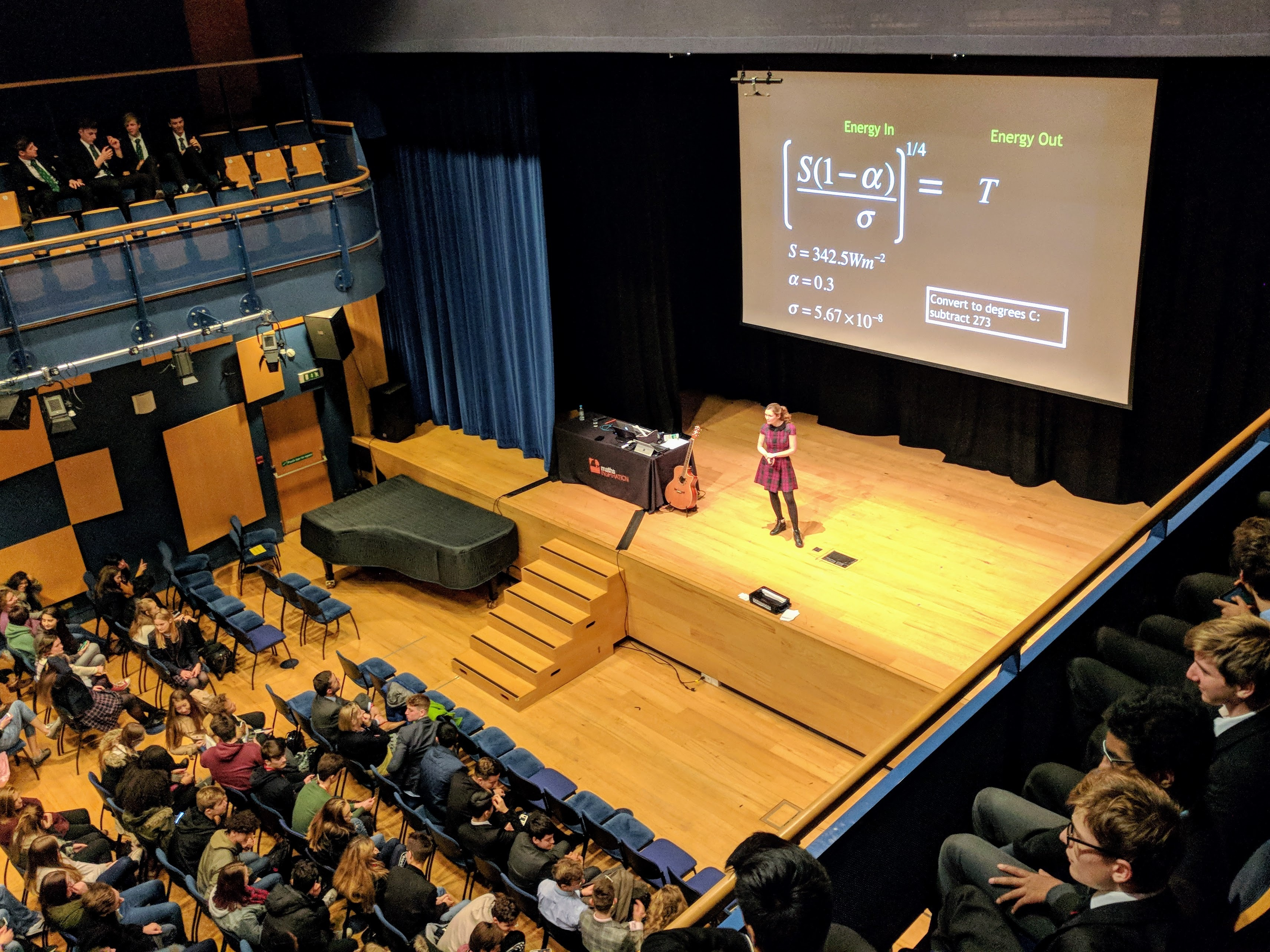Sammie talking at a Maths Inspiration event. Photo: Ben Sparks

She says there are two reasons why she believes outreach is very important for her field. “First of all, because we’re studying areas that are so affected by climate change, it’s important to talk to people about that and let them know what’s going on, because it’s so disconnected from what people do every day and what they see. The majority of people are never going to go to the Arctic or the Antarctic but what’s happening there is going to affect all of us.”

The second reason is to improve diversity in polar science. “The British Antarctic Survey had their first women go to Antarctica in the 1983, which is insane. When I was born, women weren’t allowed to go to Antarctica from the UK. I went to a fairly standard state school and the idea of being a researcher or scientist never crossed my mind, so I wanted to let people know those opportunities are out there.” She says this can be even worse for racial minorities or minority genders. But how can we improve the situation? “We are running a workshop on race and systemic bias within Arctic science, to essentially work out what we should be doing because it feels to me that there’s a lot more awareness now but we don’t really talk very much about solutions.”

One of her outreach projects particularly piqued my interest: New Atlantis. “That was kind of crazy actually. It was an immersive theatre show. The organisation New Atlantis was like a United Nations for water so it was set in the future. We were in a water crisis and the audience had to decide how we would deal with it. So were we going to rely on science, would we share the water out nicely, or were we going to have military control? And as part of that there were lots of actual scientists playing the role of future scientists. We would talk a bit about our current work but put it in the context of how things might look in 100 years or so in the future. And the audience did generally vote to leave Antarctica alone.” I wonder why that was. “I think people generally recognise that Antarctica is one of our last pristine, untouched wildernesses. Everyone loves David Attenborough, watching Frozen Planet. You look at it and think, ‘Maybe we shouldn’t touch this, and we should just let the penguins carry on as they are.'”

## From cats and dogs to stats and logs

Many people assume that in order to have a career as a mathematician, you must have been incredibly gifted from a young age. Maths is something you either get or don’t, and that reflects something immutable about you that can’t be changed. Sammie is here to tell you that this is not true, and in fact hard work and determination can be far more important than ‘natural gift’ (whatever that is). “I think I was quite good at school, but I wasn’t the best. My best friend in secondary school would get maths much more quickly than me: she would just scribble it down and be like ‘OK, I’m done!’ Meanwhile, I would take five pages to make mistakes and work out where I was going, but… I persevered.”

It was her maths teacher who told her that even though her friend seemed to have the more natural talent, her perseverance meant Sammie was more likely to get to the answer. “She would get it straight away or she would give up, whereas I would think, ‘No, I am going to get to these answers and I’ll take those five pages I need.'” But even with determination like Sammie’s, it was a while before she came to consider maths as a viable career option for her, and initially she went to university to study to become a vet. “I didn’t really know what the careers were in maths, which didn’t help. You could either become a maths teacher or an accountant and I didn’t really want to do either of those things. So I never really considered it until I actually went to vet school and realised it was not the right place for me. Then I looked more into applications like the maths of climate, cryptography and mathematical biology. I restarted my degree, and didn’t look back.”Is going to Antarctica to avoid speaking to non-mathematicians a step too far?

I think probably a lot of readers can relate to that—personally, I always found maths fun at school, but even so I struggled to envision what it would be used for in the real world. Because financial careers didn’t appeal to me, I was turned off from it until I was older and learned you could do all kinds of things with it. Applications of maths are generally not taught in school and for a lot of children, that obscures what makes it so cool. It was something Sammie had to go digging for to find out. “I did a lot of internet searches for maths careers and read a few popular maths books. I think generally universities have got a bit better at promoting where their graduates end up, so looking through prospectuses I saw people in cool jobs.” Perhaps including more about these applications that are largely unknown to the public in school curricula could go some way to encourage more children to take up maths—and maybe non-mathematicians wouldn’t keep asking us ‘What do you actually do?!’ (Just kidding. Obviously mathematicians never speak with non-mathematicians: it’s too scary!)

After choosing to study maths at the University of Exeter, Sammie then sought a PhD at the University of Reading. “I met with the person who turned out to be my supervisor, and I was actually quite concerned at the time because I didn’t know anything about ice, and he said it didn’t matter—as long as I had the maths, he would teach me the rest of it.” If any mathematicians are now feeling tempted by a career in climate science (I certainly am), that news should be reassuring. “Obviously it took a bit of work to fill in the gaps, but there was the support there because so many people come into it: it’s not like most people have a degree in glaciology.”

If there is one thing she emphasises throughout this interview, it is that climate science is for everyone. “I feel like what I do is really cool, so I want everyone to be able to do it if they want to!”# Surviving the bridge in Squid Game

In the popular Netflix drama series Squid Game, hundreds of adults in financial despair are recruited to play a sequence of games, in competition for a large cash prize. Though the games mimic those the characters played as children, ironically, these games are deadly. The players willingly participate in these games, risking their lives for the cash prize. Throughout, Squid Game makes powerful social comments on economic desperation, inequality and immobility.

If that’s insufficient to convince you to watch Squid Game, episode seven (‘VIPs’) presents an interesting survival game amenable to probabilistic analysis. Sixteen remaining players, having already beaten four deadly playground games, are now told to number themselves 1–16. Having done so, the next challenge is presented to them: the bridge survival game. How will our players fare? Inspired by this episode, we can frame this game as a stochastic process and find the survival probabilities of the players. Continue reading# Accidentally mathematical songs

## Girls & Boys by BlurThe chorus of Girls & Boys by Britpop band Blur goes:

Girls who are boys
Who like boys to be girls
Who do boys like they’re girls
Who do girls like they’re boys.
Always should be someone you really love.
If you take the sequence of ‘girls’ (G) and ‘boys’ (B), you get: GBBGBGGB. This follows the Thue–Morse sequence.

The Thue–Morse sequence is represented as a string of binary digits. The sequence can be extended by taking the Boolean complement (take the opposite to each position) of the current string and adding this to the end of the string. We start with 0, and add the Boolean complement (1) to obtain the string 01. Now we have 01, and add the Boolean complement (10) to get 0110. Repeating this a few times, we get the sequence 0110100110010110…

In fact, you could take any string of ones and zeros from the Thue–Morse sequence, and it won’t appear again until after the string finishes. For example, take the string 1101 which appears near the start. The next time this string appears is in position 14, without overlapping with the original string:

0110100110010110100101100…
In fact, you could take any series of ones and zeros in the Thue–Morse sequence, and it too won’t overlap with the same series later in the sequence.

To prove this in a hand-wavy way*, suppose that the Thue–Morse sequence is overlap-free until a string $A$ that contains an overlap appears (for example, $A$ could be 10101 as it contains overlapping 101s). But by the nature of the sequence, the Boolean complement of $A$ (01010 in our example) must have already appeared in the sequence, which means there must have been an earlier overlap. This is a contradiction, so the Thue–Morse sequence must be overlap free. QED.

Replacing 0 and 1 with G and B, we see that the start of the Thue–Morse sequence is the same as the Girls & Boys sequence. As such, we can extend the chorus:

Girls who are boys
Who like boys to be girls
Who do boys like they’re girls
Who do girls like they’re boys
Who need boys like they’re girls
Who need girls like they’re boys
Who have girls who are boys
Who have boys who are girls
Who choose boys who see girls
Who choose girls who see boys
Who meet girls who like boys
Who meet boys who like girls
Who verb girls who verb boys
Who verb boys who verb girls

Always should be someone you really love.
* Proving this fully is a little harder than this. Can you spot which bit of the proof needs a bit more work?

## Falling to Pieces by Faith No MoreIn 1990, American rock band Faith No More, released the single Falling to Pieces, from their album The Real Thing. Within the song is the verse:

From the bottom, it looks like a steep incline,
From the top, another downhill slope of mine,
But I know, the equilibrium’s there.
Which makes me think of the intermediate value theorem.

The intermediate value theorem states: ‘If $f$ is a continuous function whose domain contains the interval $[a,b]$ then it takes any given value between $f(a)$ and $f(b)$ at some point within the interval.’ A corollary of this theorem is that if you have the same conditions, then there must exist $c\in[a, b]$ such that $f(c)=(f(a)+f(b))/2$.But how does this relate to the song? Well, if we take ‘the bottom’ to be a point at $x=a$ and ‘the top’ to be a point at $x=b$, then we can define a function between them. As stated, to use the intermediate value theorem we need the function to be continuous; since it is a slope, I think it is fair to say that the surface rarely breaks—you never have a hill where suddenly halfway up you find a huge gap in the floor!

The corollary of the intermediate value theorem tells us that there must be a point exactly halfway up the vertical cross section. In other words, ‘the equilibrium’ is there; exactly as Faith No More’s conjecture stated.# On the cover: Regular tilings

Tilings have been studied for thousands of years dating back to 3000BC, and people are still finding out more and more about them now. They’ve been used in architecture for longer, and appear all over the natural world. I’m going to try and present a small subtopic within tilings that will hopefully be interesting and straightforward to understand.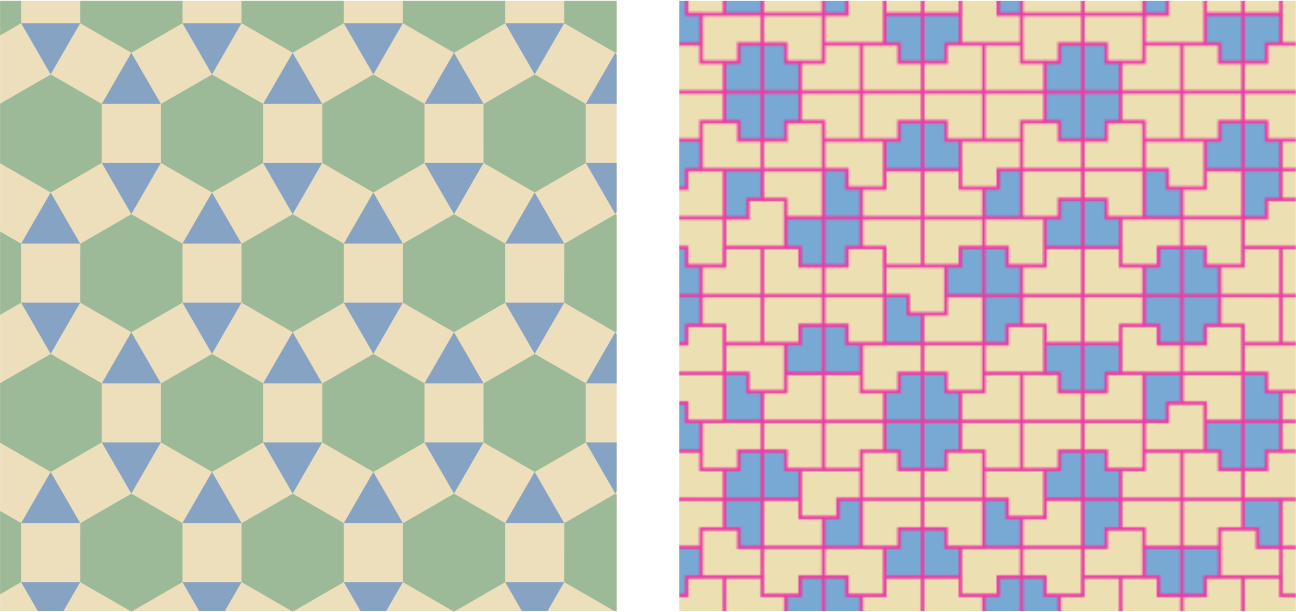The rhombitrihexagonal tiling from around 200 BC (left) and the Ammann chair tiling from AD 1977 (right). Mathematica code by Sasho Kalajdzievski, CC BY-NC-SA 3.0

## Second to the right and tile on till morning

A tiling problem, in the context of this topic, is the following: given a set of tiles (closed simple curves and their interior) can we completely cover the plane without gaps or overlaps?

The tilings which are easiest to visualise and draw are tilings by regular polygons, which I will call regular tilings here for simplicity. A lot of the results I’m going to mention can be extended to non-regular polygons, but for now let’s stick to maths which is a bit easier to deal with.

Let’s even start with the simplest kind of regular tiling: tilings that are monohedral, which means only one type of tile is used to construct it.

So how can we construct a monohedral regular tiling? Let’s start with the most common: a tiling of the plane by squares. It is easy to see how it is constructed and why it tiles the plane, simply because a square has four corners with four right angles. We know it is possible to place four squares together, edge to edge, so that all four of them have a common corner, simply because $4 \times \pi/2 = 2\pi$, which is the number of radians around a point. We can do this indefinitely so that each corner in the tiling is a corner common to four squares:A slightly more complicated tile is the regular hexagon. See if you can work out how to prove that regular hexagons tile the plane.There is only one more monohedral regular tiling of the plane, and it can be constructed using the hexagon tiling. Take a regular hexagon and draw in its three axes of reflectional symmetry. This gives us a regular tiling with only equilateral triangles. These are actually the only three monohedral regular tilings of the plane! I will prove this later on.Now I want to discuss how to construct a vertex (ie a point where at least three tiles meet) in a regular tiling. We know there are $2\pi$ radians around any vertex. Because we’re working with regular polygons, there’s a smaller number of angles that we have to choose from to make up this $2\pi$. Could we cut this down concretely?

It is a well known fact that the angle sum in a convex $n$-polygon, a polygon with $n$-sides, is $(n-2)\pi$ (to prove this, consider splitting polygons up into triangles!). From now on, when I talk about polygons I mean regular polygons.

## Counting corners

If we consider a tiling where each vertex has $r$ polygons around it, polygons with $n_1$, $n_2$, … up to $n_r$ sides respectively, then these two facts combined give us the equation $2\pi=\frac{(n_1-2)\pi}{n_1}+\cdots+\frac{(n_r-2)\pi}{n_r}.$ By cancelling $\pi$ on both sides, we get \begin{equation}\label{eq:anglesum} \frac{n_1-2}{n_1}+\cdots+\frac{n_r-2}{n_r}=2. \tag{*} \end{equation}

So any polygons around a vertex in a regular tiling have to satisfy this equation. I think this is quite cool, because now we’ve got a concrete equation that we can work with algebraically!

This equation can be used to prove there are only three monohedral regular tilings of the plane. Monohedral means that all $n_i = n$, for some integer $n$ at least 3. Then \eqref{eq:anglesum} simplifies to $\frac{n-2}{n}+\cdots+\frac{n-2}{n}=2\quad\Rightarrow\quad r\frac{n-2}{n}=2.$

Writing this in terms of $n$ gives $n=2+\frac{4}{r-2}.$ We know that $n$ is an integer, which means that $r-2$ must divide 4. This implies $r – 2 = 1, 2$, or $4$, and so $r = 3, 4,$ or $6$. This is equivalent to saying, the only monohedral regular tilings of the plane are by triangles, squares, and hexagons!

## A vertex by any other name would look the same

One property about monohedral regular tilings is that all of the vertices ‘look’ the same. This leads onto the question: what about other regular tilings where all the vertices look the same? Equation \eqref{eq:anglesum} seems like it could be quite helpful with figuring out how many different regular tilings we can form this way.

I’m going to cheat a little with this part, and just tell you that there are exactly 21 ways to satisfy \eqref{eq:anglesum}. It is also noteworthy that the order of the $n_i$’s does matter in this case because reading off the polygons around a vertex in a different order makes a different tiling. I’ve not derived or included a proof of this result because it is quite long and tedious.

There is actually a problem here. If for example we take the numbers 3, 4, 4, and 6 for the $n_i$’s. These fit the equation, but we can’t extend this arrangement of regular polygons around a vertex to a full tiling of the plane. This is because as shown below, at the black vertex we are forced to place the polygons in the order triangle-square-hexagon-square, but this is not in the order 3, 4, 4, 6. So not all vertices have the same arrangement of polygons, and this doesn’t extend to a tiling with the property we want.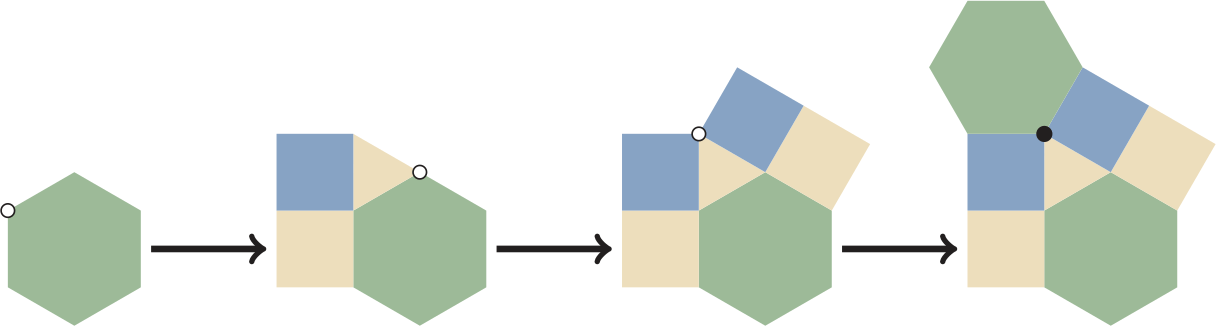Trying to build the 3, 4, 4, 6 tiling by adding polygons around each vertex (indicated with a white dot) in the order triangle-square-square-hexagon.

Just because an arrangement of polygons fits around a vertex edge-to-edge, doesn’t mean this extends to a tiling of the plane where all vertices are the same type. Have a think about why this is. For this reason, it turns out there are only 11 ways to tile the plane in the way we want to.

Showing this requires two steps. I’m not going to be super meticulous here and do this for all 21 different options, but I’ll do an example for each step.

1. We need to show that for 10 types of vertices, we can’t extend from the region around the starting vertex to a tiling of the plane, like we did for the numbers 3, 4, 4, 6 above.
2. We need to show that the remaining 11 types of vertices actually lead to tilings of the plane. We already showed this for the monohedral tilings, and in the picture below I show it for constructing a tiling with polygons 3, 6, 3, 6 from the monohedral triangle tiling:Constructing tiling with polygons 3, 6, 3, 6 starting with the monohedral tiling by equilateral triangles. Shaded regions are where the hexagons come from.

These 11 tilings are known as the Archimedean tilings. So now we’ve shown that there are only 11 regular tilings where each vertex has the same arrangement of polygons around it! This, to me, is a good example of why maths is so interesting. We started with a problem that appeared to have an infinite number of solutions and managed to use only basic high school techniques and knowledge to deduce that there were only 11 different solutions instead!

## Beyond Archimedean tilings

The Archimedean tilings all have another property, and that is that they are periodic, invariant under translations in two non-collinear directions. I find periodic tilings really interesting as it’s fairly easy to see how they’re constructed.

I also study aperiodic tilings, tilings that have no translational invariance. These can be studied using other branches of maths, such as topology. There is an object called the tiling space which is defined as all the translations of a tiling. For the grid unit square tiling, the tiling space is a torus. This is because the tiling is equivalent under translation by one tile horizontally or vertically, so opposite edges of a square are identified, which gives a flat torus.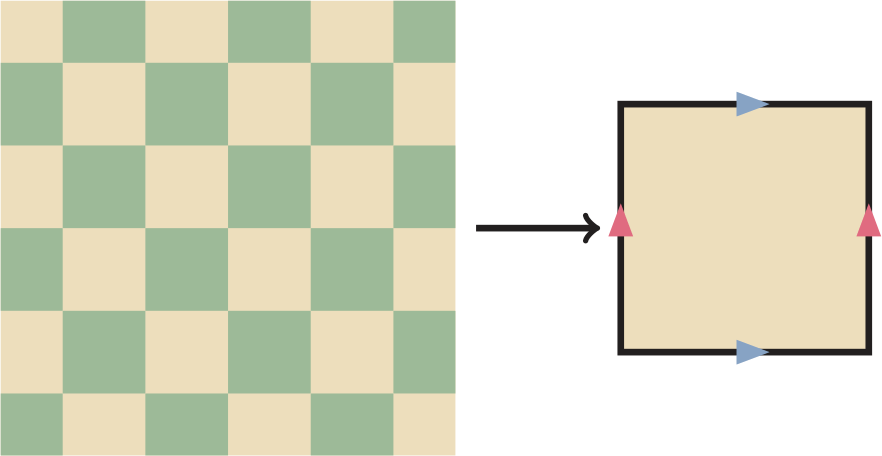The tiling space of every periodic tiling is always the torus, so this topological approach isn’t so helpful for periodic tilings, but it is for aperiodic tilings. If you’re interested to learn more, a good article to introduce the subject is A primer of substitution tilings on the Euclidean plane by Natalie Frank. And, if you’re interested in geometry and tilings, then consider reading the book Tilings and patterns by Branko Grünbaum and Geoffrey Colin Shephard. You could also look into Platonic solids, which are an introduction to some of these ideas in three dimensions.# The power of curly brackets

## 🍎 Newton

One of the most famous formulas in physics is Newton’s second law, $\boldsymbol{F} = m \boldsymbol{a}.$ It is named after Isaac Newton (1643–1727), who in all likelihood was never actually hit on the head by any apples, and states that ‘force equals mass times acceleration’. It shines in its simplicity, but contemporary mathematical physicists would much rather write it as
$\frac{\mathrm{d} z}{\mathrm{d}t} = \{H,z\} .$
In this formula, $z$ can be any quantity related to the system and $H$ is the Hamilton function, which represents the total energy of the system. But what is this strange bracket? And why would any sane person write a simple idea like Newton’s second law in such an obscure way?

There are a few possible answers to this last question. I could start singing praise for some abstract geometric beauty, but it also provides an easy explanation of Emmy Noether’s famous theorem on the relation between symmetries and conserved quantities. A conserved quantity, as the name suggests, is something that does not change. The most common example is probably the conservation of energy in physics, but there can be many other conserved quantities.

Noether’s (first) theorem states that a system has a conserved quantity if and only if it possesses a related symmetry. Translation symmetry corresponds to conservation of (linear) momentum, for example, and rotational symmetry to angular momentum.

## 1️⃣🇮🇯🇰 Hamilton

Let’s consider a physical system consisting of one point particle of mass $m$ moving through space. The state of the system is given by its position $\boldsymbol{x} = (x_1,x_2,x_3)$ and momentum $\boldsymbol{p} = (p_1,p_2,p_3)$. In mechanics, momentum is simply the product of mass and velocity, $\boldsymbol{p} = m \boldsymbol{v}$, so you could also say the state is given by position and velocity. But it turns out that using momentum leads to more elegant mathematics. If we know the state of the system at some point in time, then the states at all future (and past) times are determined by Newton’s second law.

Suppose we can write the potential energy of the system as a function $V(\boldsymbol{x})$ of the position. Then the force on the particle is given by minus the gradient of this function, $F(\boldsymbol{x}) = -\boldsymbol{\nabla} V(\boldsymbol{x})$, so Newton’s second law can be written as
$\frac{\mathrm{d}^2 \boldsymbol{x}}{\mathrm{d}t^2} = – \frac{1}{m} \boldsymbol{\mathsf{\nabla}} V(\boldsymbol{x}),$
or, written in components,
$\frac{\mathrm{d}^2 x_i}{\mathrm{d}t^2} = – \frac{1}{m} \frac{\partial V(\boldsymbol{x})}{\partial x_i}.$
Since the kinetic energy of the particle is $|\boldsymbol{p}|^2/(2m)$, the total energy will be given by
$H(\boldsymbol{x},\boldsymbol{p}) = \frac{1}{2m} |\boldsymbol{p}|^2 + V(\boldsymbol{x}) .$

This is called the Hamilton function, after William Rowan Hamilton (1805–1865), who is also famous as the inventor/discoverer of the quaternions. One reason why this function deserves its special name, is that it gives the equations of motion in a very satisfying way. The time derivatives of position and momentum are, up to sign, equal to the partial derivatives of $H$: $\frac{\mathrm{d} \boldsymbol{x}}{\mathrm{d}t} = \frac{\partial H}{\partial \boldsymbol{p}} \qquad\text{and}\qquad \frac{\mathrm{d} \boldsymbol{p}}{\mathrm{d}t} = -\frac{\partial H}{\partial \boldsymbol{x}}.$ We call these the ‘equations of motion’. By combining the two equations of motion, you can find an expression for the acceleration $\mathrm{d}^2 \boldsymbol{x}/\mathrm{d}t^2$. You can check that this again gives Newton’s second law, so these equations really do describe the motion of the system.

For our choice of Hamilton function, the first equation of motion will simply be $\mathrm{d} \boldsymbol{x}/\mathrm{d}t = \boldsymbol{p}/m$, but we prefer to write it in the form above. It is not just pleasing to have both equations of motion look so similar, but it also helps us find the time derivative of $H$ itself. By the chain rule we have $\frac{\mathrm{d} H}{\mathrm{d}t} = \frac{\partial H}{\partial \boldsymbol{x}} \cdot \frac{\mathrm{d} \boldsymbol{x}}{\mathrm{d}t} + \frac{\partial H}{\partial \boldsymbol{p}} \cdot \frac{\mathrm{d} \boldsymbol{p}}{\mathrm{d}t},$ where the dot denotes the scalar product between vectors. If you prefer to write out the components, you can expand the first term as $\frac{\partial H}{\partial \boldsymbol{x}} \cdot \frac{\mathrm{d} \boldsymbol{x}}{\mathrm{d}t} = \frac{\partial H}{\partial x_1} \frac{\mathrm{d} x_1}{\mathrm{d}t} + \frac{\partial H}{\partial x_2} \frac{\mathrm{d} x_2}{\mathrm{d}t} + \frac{\partial H}{\partial x_3} \frac{\mathrm{d} x_3}{\mathrm{d}t}$ and the second term in a similar way. Using the equations of motion we can see the two terms in the expression for $\mathrm{d} H/\mathrm{d}t$ cancel: $\frac{\mathrm{d} H}{\mathrm{d}t} = \frac{\partial H}{\partial \boldsymbol{x}} \cdot \frac{\partial H}{\partial \boldsymbol{p}} – \frac{\partial H}{\partial \boldsymbol{p}}\cdot \frac{\partial H}{\partial \boldsymbol{x}} = 0,$ so we can call $H$ a conserved quantity, because it doesn’t change in time. Hence any system that fits into this Hamiltonian framework automatically satisfies conservation of energy.

## 🔭 Kepler

When thinking about physics, it is always useful to have a particular system in mind. Consider for example a planet orbiting the sun (where we approximate both by point masses and assume that the sun is fixed at the origin). This is known as the Kepler system, after Johannes Kepler (1571–1630), who figured out the laws of planetary motion long before Newton came up with a theory of gravity.

The Kepler system is governed by the Hamilton function, $H(\boldsymbol{x},\boldsymbol{p}) = \frac{1}{2m} |\boldsymbol{p}|^2 – \frac{1}{|\boldsymbol{x}|}.$ Its equations of motion are $\frac{\mathrm{d} \boldsymbol{x}}{\mathrm{d}t} = \boldsymbol{p} \qquad\text{and}\qquad \frac{\mathrm{d} \boldsymbol{p}}{\mathrm{d}t} = \frac{-\widehat{\boldsymbol{x}}}{|\boldsymbol{x}|^2},$ where $\widehat{\boldsymbol{x}}$ denotes the unit vector in the direction of $\boldsymbol{x}$. This is Newton’s second law with the inverse square central force $F(\boldsymbol{x}) = -\frac{\widehat{\boldsymbol{x}}}{|\boldsymbol{x}|^2}.$

If the planet is moving relatively slowly, its motion will consist of repeated orbits, tracing out an ellipse. If it is speeding, then its orbit will either be a parabola or a hyperbola, so it will approach the sun only once before rushing off into interstellar space. Let’s ignore the possibility of such a speedy galactic nomad and assume that we are dealing with an elliptic orbit.

The Kepler system has some notable properties. One of these is that it is rotationally symmetric (see below). This can be seen from the formula for the Hamilton function: it only depends on the lengths of $\boldsymbol{x}$ and $\boldsymbol{p}$, not their directions, so it will not change if we rotate these vectors.

The diagram below shows a planet (blue) orbiting the sun (yellow), tracing an ellipse. It is shown near its closest point to the sun, together with its velocity vector.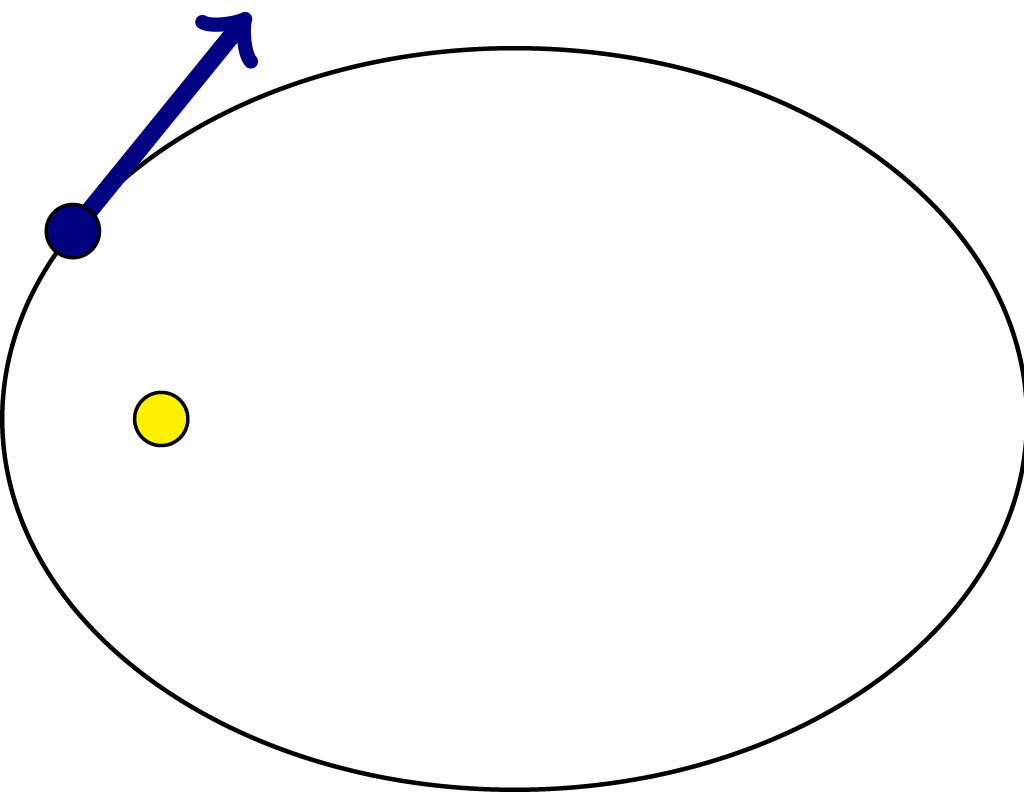Rotational symmetry means that if we instantly rotate both the planet and its velocity vector through some angle around the sun, then the new orbit will be the same ellipse as before, rotated by the same angle.The conserved quantity corresponding to rotational symmetry is the angular momentum $\boldsymbol{L} = \boldsymbol{x} \times \boldsymbol{p}$ (see below). We can check this by verifying that its time derivative is zero. Using the product rule we find \begin{align*} \frac{\mathrm{d} \boldsymbol{L}}{\mathrm{d}t} &= \frac{\mathrm{d} \boldsymbol{x}}{\mathrm{d}t} \times \boldsymbol{p} + \boldsymbol{x} \times \frac{\mathrm{d} \boldsymbol{p}}{\mathrm{d}t} \\ &= \boldsymbol{p} \times \boldsymbol{p} + \boldsymbol{x} \times \frac{-\widehat{\boldsymbol{x}}}{|\boldsymbol{x}|^2}, \end{align*} which is zero because the cross product of parallel vectors is always zero. Hence $\boldsymbol{L}$ is indeed a conserved quantity.

Angular momentum ($\boldsymbol{L} = \boldsymbol{x} \times \boldsymbol{p}$) is a measure of how much an object is spinning (around a reference point). It increases both when the speed of rotation increases and when the distance to the reference point grows.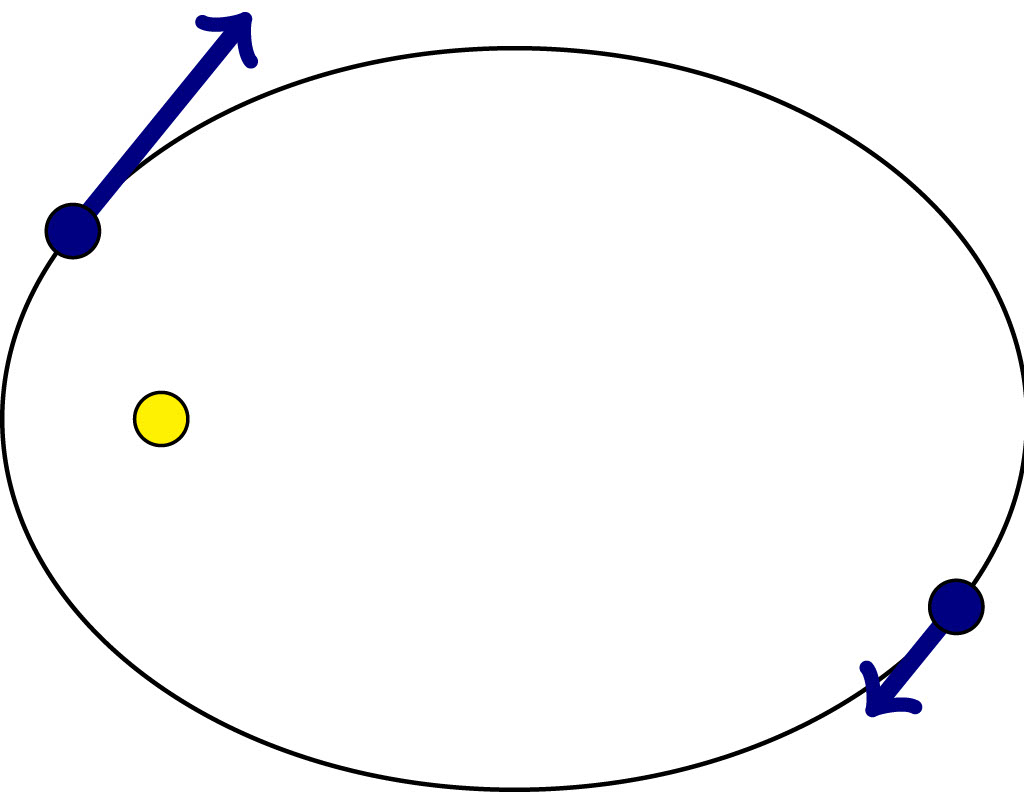Conservation of angular momentum implies that the planet moves slower when it is further from the the sun. A terrestrial example of conservation of angular momentum can be observed when a figure skater performs a pirouette. They will spin more quickly when they move some of their mass closer to the rotation axis by bringing in their arms.Our observations on the Kepler system illustrate Noether’s theorem, which states that symmetries and conserved quantities are in one-to-one correspondence. In particular, if a system is rotationally symmetric, then it conserves angular momentum. Other systems may have different symmetries. If a system has translation symmetry (eg billiards on an unbounded table) it conserves the total linear momentum. Even conservation of energy fits into this picture. It corresponds to the time translation symmetry: the laws of physics don’t change over time.

To prove Noether’s theorem, we need one more item in our toolbox.

## {🐟,🐠} Poisson

Earlier we checked that $H$ is always a conserved by calculating its time derivative. What if we want to know the time derivative of some other function $z$ of position and momentum? We can calculate \begin{align*} \frac{\mathrm{d} z}{\mathrm{d}t} &= \frac{\partial z}{\partial \boldsymbol{x}} \cdot \frac{\mathrm{d} \boldsymbol{x}}{\mathrm{d}t} + \frac{\partial z}{\partial \boldsymbol{p}} \cdot \frac{\mathrm{d} \boldsymbol{p}}{\mathrm{d}t} \\ &= \frac{\partial z}{\partial \boldsymbol{x}} \cdot \frac{\partial H}{\partial \boldsymbol{p}} – \frac{\partial z}{\partial \boldsymbol{p}} \cdot \frac{\partial H}{\partial \boldsymbol{x}}. \end{align*}

The expression in the last line is usually written as: $\{H,z\} := \frac{\partial H}{\partial \boldsymbol{p}} \cdot \frac{\partial z}{\partial \boldsymbol{x}} – \frac{\partial H}{\partial \boldsymbol{x}} \cdot \frac{\partial z}{\partial \boldsymbol{p}} .$ This curly bracket is called the Poisson bracket, after Siméon Denis Poisson (1781–1840), whose name sounds a lot less posh once you remember that ‘poisson’ is French for ‘fish’.

With this definition, we obtain our brackety formula, $\frac{\mathrm{d} z}{\mathrm{d}t} = \{H,z\} ,$ as a reformulation of the Hamiltonian equations of motion.

We can also go the other way and recover the Hamiltonian equations of motion from the brackety formula. Since $z$ can be any function of position and momentum, we can choose to set it equal to a component of either position or momentum and find \begin{align*} \frac{\mathrm{d} x_i}{\mathrm{d}t} &= \{H,x_i\} = \frac{\partial H}{\partial p_i} &&\text{and}& \frac{\mathrm{d} p_i}{\mathrm{d}t} &= \{H,p_i\} = -\frac{\partial H}{\partial x_i}. \end{align*}

We have now established that $\mathrm{d} z/\mathrm{d}t = \{H,z\}$ is equivalent to the Hamiltonian equations of motion, but why do we care about Poisson brackets? Well, they have some interesting properties:

1.  A function $z$ of position and momentum is a conserved quantity of the system with Hamilton function $H$ if and only if $\{H,z\} = 0$. This follows immediately from the brackety formula: the vanishing of the Poisson bracket is equivalent to $\mathrm{d} z/\mathrm{d}t = 0$.
2. The Poisson bracket is skew-symmetric, meaning that if we swap around its entries, we get the same result but with a minus sign: $\{z,u\} = -\{u,z\}$ for any two functions $u$ and $z$ of position and momentum.

There are additional properties of the Poisson bracket which make sure that the identification of a time derivative with a bracket makes sense, but we won’t go into those technicalities here. Instead, let’s spin our attention towards Noether.

## 🔄 $\Leftrightarrow$💃🏿 Noether

Emmy Noether (1882–1935) was a mathematician of many talents. Much of her work was in abstract algebra, but she is most famous for her theorem stating that conserved quantities of a system are in one-to-one correspondence with its symmetries. She counts as one of the top mathematicians of the interwar period, a status she managed to achieve in the face of cruel discrimination because of her gender and descent. At the University of Göttingen, Germany, where she spent most of her career, she was refused a paid position, despite strong support from her colleagues David Hilbert and Felix Klein. In 1933, she emigrated to the US to escape the Nazi regime.

Let’s put aside the grim history and step into Noether’s mathematical footsteps to find out what symmetries have to do with conserved quantities. Consider two Hamilton functions $H$ and $I$ and the corresponding dynamical systems, \begin{align} \frac{\mathrm{d} z}{\mathrm{d}t} &= \{H,z\} , \label{H}\tag{$H$}\\ \frac{\mathrm{d} z}{\mathrm{d}t} &= \{I,z\} . \label{I}\tag{$I$} \end{align}

In the Kepler problem, for example, the system labelled \eqref{H} would describe the physical motion of a planet and the one labelled \eqref{I} a rotation around the sun. The Hamilton function for a rotation is a component of the angular momentum vector, so in this example we would take $I$ equal to a component of $\boldsymbol{L} = \boldsymbol{x} \times \boldsymbol{p}$.

Now what does it mean for \eqref{I} to be a symmetry of the system \eqref{H}? It means that the motion of \eqref{I} does not change the equation \eqref{H}. Since the dynamics of a system is fully encoded by its Hamilton function, this is equivalent to saying that the system \eqref{I} does not change the Hamilton function $H$. Hence $\text{\eqref{I} is a symmetry of \eqref{H}} \quad \iff \quad \text{H is a conserved quantity of \eqref{I}} .$

We can use property (A) to express this in terms of a Poisson bracket: $\text{\eqref{I} is a symmetry of \eqref{H}} \quad \iff \quad \{I,H\}= 0 .$ Next we use property (B): the Poisson bracket is skew-symmetric, hence $\text{\eqref{I} is a symmetry of \eqref{H}} \quad \iff \quad \{H,I\}= 0 .$ Or, using property (A) once again: $\text{\eqref{I} is a symmetry of \eqref{H}} \quad \iff \quad \text{I is a conserved quantity of \eqref{H}} .$ This is essentially the statement of Noether’s first theorem: the symmetries of a system are related to its conserved quantities.

There is an important thing that we have swept under the rug in this derivation. Not every possible symmetry is generated by a Hamilton function. Hence the correct formulation of Noether’s theorem is that conserved quantities are in one-to-one correspondence with Hamiltonian symmetries. This issue disappears when, instead of Hamiltonian mechanics, we consider Lagrangian mechanics, which is based on the calculus of variations. Within that framework a natural notion of symmetry leads to a one-to-one correspondence between symmetries and conserved quantities.

In fact, Noether’s original paper dealt with the Lagrangian perspective. It included not just the case of mechanics, but also field theory, which deals with partial differential equations. Her main motivation was to understand conservation of energy in Einstein’s theory of gravity. This is a surprisingly subtle problem, because general relativity has an infinite number of symmetries.

When a system has an infinite number of symmetries, the conserved quantities produced by Noether’s first theorem are trivial in some sense. For example, a function which maps position and momentum to a constant would be a trivial conserved quantity: it does not change in time, but that fact does not tell us anything about the dynamical system. Noether’s second theorem is relevant to these systems with infinitely many symmetries. Roughly speaking, it says that if a system has an infinite number of symmetries, then the equations of motion must have a certain redundancy to it: some of the information contained in one of the equations of motion will also be contained in the others.

## 💡 Noether’s legacy

It was known before Noether’s time that conserved quantities are related to symmetries. And while her paper was the first one to make this connection precise, her main breakthrough was the lesser known second theorem. Noether’s insights were warmly welcomed by mathematical physicists of the time, including Albert Einstein himself, and are still a key part of modern physics. The power of Noether’s theorems lies in their generality: they apply to any system with the relevant kind of symmetries, and to prove them you don’t need to know the particulars of the system at hand.

Similarly, Poisson brackets allow us to capture essential features of physics with an equation that takes the same form no matter what system it describes. Instead of having to work out all the forces between interacting objects, all you need to put into this framework is the total energy in the form of the Hamilton function. It’s no wonder that mathematical physicists often prefer $\mathrm{d} z/\mathrm{d}t = \{H,z\}$ over $\boldsymbol{F} = m\boldsymbol{a}$.When was the last time you used a satnav in your car? Tagged your location on a social media post? Or used maps on your phone to figure out where to go? Allow me to introduce a significant figure to you, without whom none of those things would be possible.

Gladys West was a pioneer in geodesy—the branch of mathematics that deals with the precise shape and area of the Earth. Her work was critical in the development of global positioning systems (GPS). Specifically, her work was used to put together extremely precise models of the Earth’s shape, enabling GPS technology which impacts every single one of us on the planet.# The hidden harmonies of Hamming distanceWe think about the concept of distance all the time—how far is it to go visit a friend, is my phone close enough to the router to get wifi?—but in mathematics there are many ways of measuring the distance between things.

Mathematics is sometimes thought to be a world of dense, dry formulae and esoteric logic, but its structures, especially when paired with modern computing, can generate images of arresting complexity like the one above. The goal of this article is to introduce and explore these images, which arise from attempts to visualise a notion of distance on the integers called Hamming distance.

A distance function assigns a non-negative number to any pair of objects to serve as a measure of how far apart they are. In order to qualify as a distance, such an assignment needs three key features. First, the distance from an object to itself is zero. Second, the distance from object $x$ to object $y$ is always the same as the distance from object $y$ to object $x$. Finally, a path from one object to another that goes through a third is never shorter than the direct path between the two objects.

More formally, if we denote the distance from $x$ to $y$ by $D(x,y)$, then for any object $z$, $$D(x,y)\leq D(x,z)+D(z,y).$$ In what follows, we will consider two different ways of measuring the distance between positive integers.

## Euclidean distance and Hamming distance

The most common way to measure the distance between two numbers is using Euclidean distance, with which you may already be familiar. Given two numbers $x$ and $y$, the Euclidean distance between them, expressed as $E(x,y)$, is simply $\vert x-y\vert$.

In the mid-twentieth century, the American mathematician Richard Hamming developed the Hamming distance to measure the distance between sequences of zeros and ones by counting the number of positions in which the sequences differ, a measure that has the three features necessary for a distance. So, for example, the Hamming distance between 10110 and 11110 is 1 as they only differ in the second position from the left—these strings are close.

Working in the early days of computer science, Hamming used this distance measure in his analysis of the effectiveness of error detection and correction in the transmission of messages encoded by strings of zeros and ones. The number of errors an encoding could correct was related to how ‘spread out’ the space of code words was as measured by Hamming distance. Hamming distance can also be thought of as a distance on the integers by using binary representations.

For example, \begin{align*} 22 & = 1 {\color{gray}\times 2}^4 + 0 {\color{gray}\times 2}^3 + 1 {\color{gray}\times 2}^2 + 1 {\color{gray}\times 2}^1 + 0 {\color{gray}\times 2}^0 = 10110_2,\\ 30 & = 1 {\color{gray}\times 2}^4 + 1 {\color{gray}\times 2}^3 + 1 {\color{gray}\times 2}^2 + 1 {\color{gray}\times 2}^1 + 0 {\color{gray}\times 2}^0 = 11110_2. \end{align*} Here, the subscript $2$ indicates that the number is in base 2 rather than base 10. Writing $H(x,y)$ for the Hamming distance between two positive integers $x$ and $y$, $H(22,30)=1$ because the binary representations of 30 and 22 differ in one position. Of course, the Euclidean distance is $E(22,30) = \vert 22-30\vert=8$.

The binary representation of the number six, $110_2$, has only three digits, but we can measure the distance from 6 to a number like 22 whose binary representation has five digits by padding with extra zeros on the left, which has no effect on the underlying number. So $6 = 110_2=00110_2$, and $H(6,22) = H(00110_2, 10110_2) = 1.$ Hamming distance clearly measures distance between positive integers quite differently than the standard Euclidean distance does. For example, $H(22,25)=4$, so 22 is much further from 25 than it is from 6 as measured by Hamming distance, but 22 is much closer to 25 than to 6 on the number line. While we can ‘see’ the Euclidean distance between two numbers in their separation on the number line, it is not immediately clear how or whether we can visualise Hamming distance.

## Euclidean distance in a square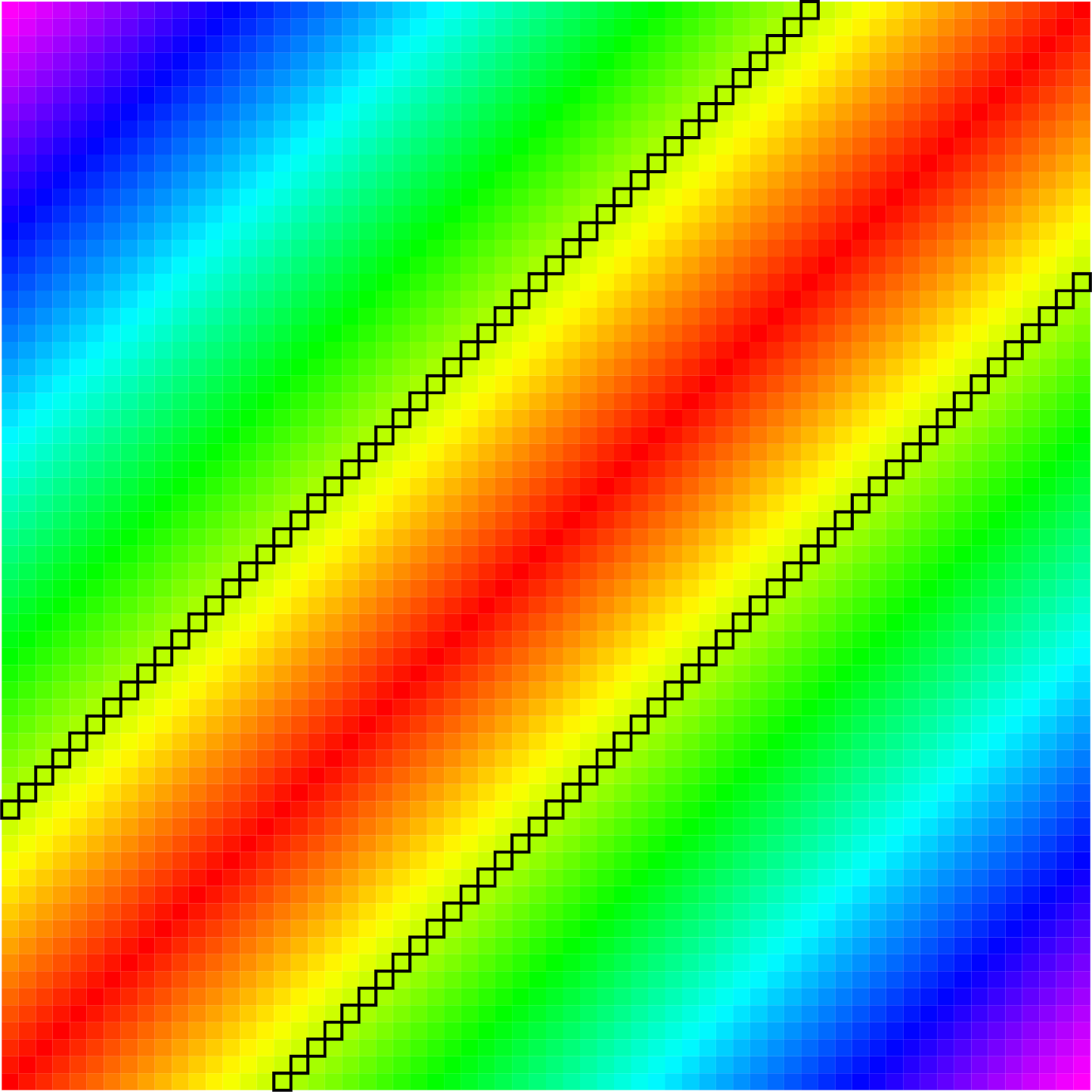Euclidean distance visualised in a square. If $E(i,j)=E(i’,j’)$ then $(i,j)$ and $(i’,j’)$ are the same colour; cells with $E(i,j) =16$ are outlined.

Distance is a function of two variables, so let us try to visualise $E(i,j)$ and $H(i,j)$ as the pair $(i,j)$ ranges over a grid of integer pairs. We’ll define $B_n$ to be the set of positive integers whose binary representation contains $n$ or fewer digits, so ${B_n = \left\{ 0,1,\dots, 2^n-1\right\}}$.

Our first strategy to visualise Euclidean and Hamming distance is to use the grid $G_n = \{(i,j)\mid i,j\in B_n \}$ as our canvas and give each different value of $E(i,j)$ a different colour. For Euclidean distance, this gives a fairly simple picture. Points $(i,j)$ that satisfy $E(i,j)=k$ lie either on the line $y=x+k$ or $y=x-k$, and so the points whose coordinates are at Euclidean distance $k$ consist of two parallel lines. On the right, the squares are $128\times 128$ cells, with the cell at position $(i,j)$ corresponding to that point in the grid.

While this picture is fairly simple, there are some features worth noting. First, the image is symmetric about the line $y=x$ because $E(x,y) = E(y,x)$, a symmetry that would be present for any distance measure. Second, the image is unchanged if we translate along the line $y=x$ because for any $z$, \begin{equation} \label{EuclideanSymmetry}\tag{🟢} E(x+z, y+z) = E(x,y). \end{equation}

## Hamming distance in a square

Using the same strategy to visualise Hamming distance produces more interesting images. The picture below shows a few colourings of the $128 \times 128$ grid $G_7$ using different values of the Hamming distance between cell coordinates. Colouring all the points in the grid $G_9$ according to the Hamming distance between their coordinates gives the image at the start of the article.

One thing to notice is that there are only $n$ possible values for the Hamming distance between two points in $B_n$, so there are only $n$ colours used in the grid, whereas there are $2^n$ possible values for the Euclidean distance between two such points. What is interesting about this picture is the nested structure of the grids $G_n$—the image is a fractal in that the same patterns recur at progressively smaller scales.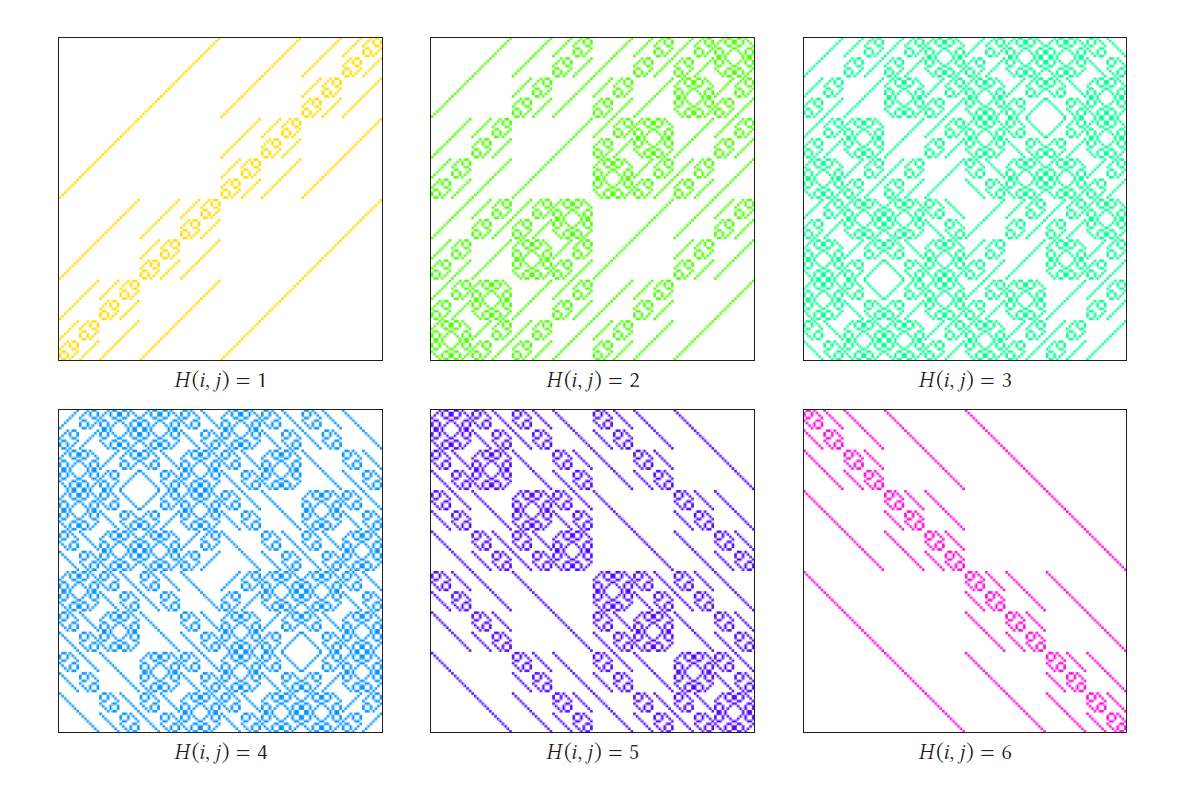The fractal looks like a quilt made of squares of similar structure. Moving from a point $(i,j)$ in some sub-square of the grid to a similarly positioned point in another sub-square involves translating one or both components by a power of two. To explore this, let’s use the notation $x+A$ for the set of numbers  in the set $A$ shifted by $x$. So formally, $x+A = \{x+a\mid a\in A \}$, and, for example, $3+\{8,9,10\} = \{11,12,13\}$. This notation allows us to recognise $B_{n}$ as consisting of $B_{n-1}$ together with $2^{n-1} + B_{n-1}$. For example: $B_2 = \left\{ 0,1,2,3 \right\} \quad \textrm{and} \quad B_3 = \left\{ 0,1,2,3,0+2^2, 1+2^2, 2+2^2, 3+2^2 \right\}.$ This means that the grid $G_{n}$ is built from four quadrants that are shifts of $G_{n-1}$ (depicted below right).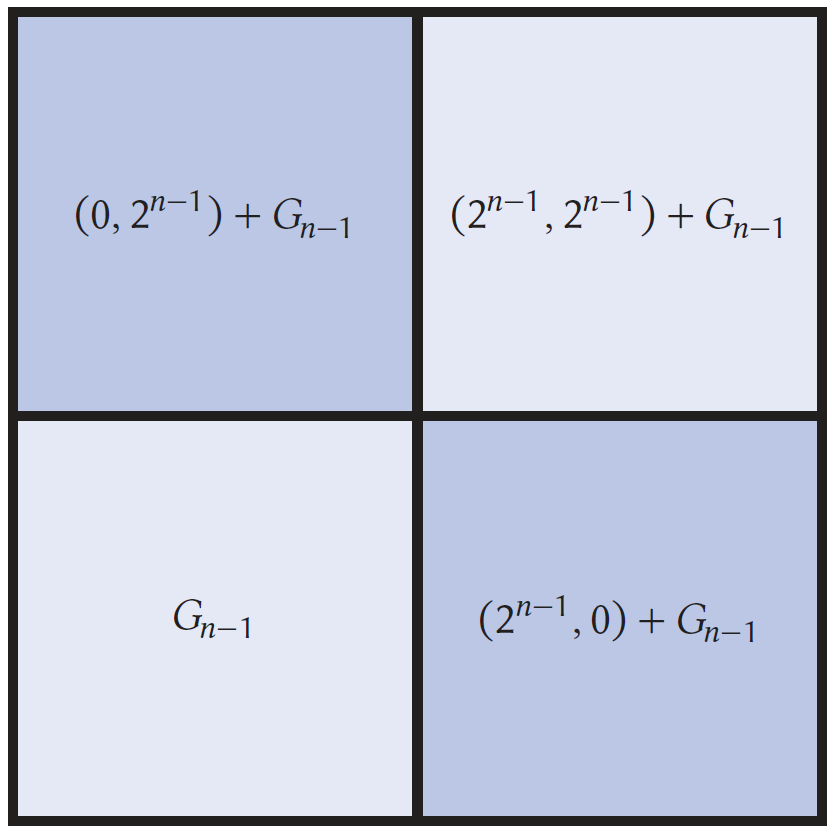If $i\in B_{n-1}$, then the binary representation of $i+2^{n-1}$ is identical to that of $i$ except for having a 1 in the $2^{n-1}$th place instead of a 0. It follows that if $j$ is also in $B_{n-1}$, then $i+2^{n-1}$ and $j$ differ in all the same places $i$ and $j$ do, with an additional difference in the $2^{n-1}$th place. If, for example, $i=01101_2$, $j=00111_2$ where $i,j\in B_5$, then $i+2^5=101101_2$. Stated more precisely: \begin{equation} \label{HammingShiftEquation}\tag{⭐} H(i+2^{n-1}, j) = H(i,j) + 1. \end{equation} This means that the cells in the lower right quadrant will have a colour value of exactly one more than the cell in the corresponding position in the lower left quadrant. A similar calculation shows that \begin{equation} \label{HammingShiftEquation2}\tag{🔺} H(i, j+2^{n-1}) = H(i,j) + 1. \end{equation} In a similar way, the upper left quadrant has the same relationship to the lower left quadrant as the lower right quadrant does. In particular, the upper left and lower right quadrants have identical colouring. If $i,j\in B_{n-1}$, then $i+2^{n-1}$ and $j+2^{n-1}$ both have a 1 in the $2^{n-1}$th place and differ in precisely the same places as $i$ and $j$. If we again take $i=01101_2$, $j=00111_2\in B_5$, then $i+2^5=101101_2$ and $j+2^5=100111_2$. That is, \begin{equation} \label{HammingShiftEquation3}\tag{💜} H(i+2^{n-1}, j+2^{n-1}) = H(i,j). \end{equation} This means the colouring of the upper right block will be identical to that of the lower left block. Since this block structure holds for $n=1,2,\dots$, we can build the Hamming fractal starting with $G_1$. The first few steps are shown below:The nested structure illustrated here causes the fractal nature of the grids $G_n$ to become increasingly apparent as $n\to \infty$. For larger values of $n$, the images contain finer detail and more complex self-similarity. In fact,  Equations (⭐) to  (💜) which cause the structure in these images, have generalisations that involve a type of addition called the XOR sum.

## The block structure and XOR sums

Hamming distance counts the number of positions in which the binary representations of $i$ and $j$ differ and is closely related to the binary operation of XOR addition. The XOR sum of two natural numbers $i$ and $j$, which we will denote $i\oplus j$, is the natural number whose binary representation has a 1 in any position where the binary representations of $i$ and $j$ differ and a 0 in any position where they are the same. For example, $\begin{array}{l l | l l} & 61 & & 111101_2 \\ \oplus & 15 & \oplus & 001111_2 \\ \hline & 50 & & 110010_2 \end{array}$ If we use the notation $\Vert i \Vert$ for the number of ones in the binary representation of $i$, then $\Vert i \Vert = H(0,i)$. The binary representation of $i\oplus j$ has as many 1s as positions where the binary representations of $i$ and $j$ differ, so $H(i,j) = \Vert i\oplus j \Vert$. Because the binary representation of $2^{n-1}$ contains all zeros except for a one in the $2^{n-1}$ position, for any $i$, $i\oplus 2^{n-1}$ will have an identical binary representation to $i$ except in the $2^{n-1}$ position, where it will differ.

Another way of thinking of the block structure in the images in the previous section is in terms of the $\oplus$ operation. If $i,j\in B_{n-1}$, so both $i$ and $j$ have zeros to the left of the $2^{n-2}$th place in their binary representations, then adding $2^{n-1}$ to either number affects their binary representations only by adding a one in the $2^{n-1}$th place. That is, equations (⭐) and (🔺) can be generalised if $i+2^{n-1}$ is replaced by $i\oplus 2^{n-1}$, so that it becomes $\Vert (i\oplus 2^{n-1}) \oplus j \Vert = \Vert i\oplus j \Vert+1\quad \textrm{and} \quad \Vert i \oplus (j\oplus2^{n-1}) \Vert =\Vert i\oplus j \Vert+1.$ Moreover equation (💜) generalises to become $\Vert (i\oplus2^{n-1})\oplus (j\oplus2^{n-1}) \Vert =\Vert i+j \Vert$.

Equations (⭐) to (💜), as well as giving rise to this nested structure, can also be generalised using the XOR sum to explain more intricate symmetries of this image. Suppose the numbers $i$ and $j$ agree at some position of their binary expansions like $i =01\mathbf{1}01_2$ and $j=10\mathbf{1}10_2$, which agree in the third position. Then ‘$\oplus$-ing’ the same number to both doesn’t change this agreement. If, for example, $a=10100_2$, then $i\oplus a = 11\mathbf{0}01_2$ and $j\oplus a = 00\mathbf{0}11_2$ both also agree in this position.

If, on the other hand, $i$ and $j$ disagree at the some position of their binary expansions like $i =01\mathbf{0}01_2$ and $j=10\mathbf{1}10_2$, which disagree in the third position, then ‘$\oplus$-ing’ the same number to both doesn’t change this disagreement either. Here, $i\oplus a = 11\mathbf{1}01_2$ and $j\oplus a = 00\mathbf{0}11_2$ both also disagree in this position. Thus, the binary expansions of $i\oplus a$ and $j \oplus a$ differ in precisely the same positions as the binary expansions of $i$ and $j$, so \begin{equation} \label{HammingSymmetry}\tag{📐} H(i\oplus a, j\oplus a) = H(i,j). \end{equation} Comparing this to equation (🟢), we see that the operation $\oplus$ is to Hamming distance much as the operation of ordinary addition is to Euclidean distance.

## Visualisations on a circle

We can get an alternative visualisation by identifying the points of $B_n$ with equally spaced points around a circle, and connecting points that are a given distance apart.

We place the number 0 at the three o’clock position on the circle and spread the numbers $0,1,\dots, 2^n-1$ evenly around the circle anticlockwise. If we connect points that correspond to numbers that are Hamming distance $k$ from one another, we get some interesting images. The image on the right corresponds to $n=4$ and $k=2$ and the image below to $n=6$ and $k=3$.

The relationship in equation (📐) illuminates many symmetries of these images. If we ‘$\oplus$-shift’ all the points on the circle, that is, if we move every vertex $i$ to the vertex $i\oplus a$ for some $a$, then we don’t change any of the connections because such a shift doesn’t change Hamming distance.

For example, if $a=2^n-1$, the binary representation of $a$ consists of $n$ ones, and the binary representation $x\oplus a$ has ones precisely where that of $x$ has zeros, and has zeros precisely where that of $x$ has ones. That is, $x\oplus a = a-x$, and the image is symmetric with respect to the interchange of $x$ and $a-x$, which amounts to a reflection over a line through the centre of the circle.

In the image (below left) purple arrows connect each point $x$ with the point $a-x$. Choosing a different value of $a$, say $a=3$, then interchanging $x$ and $x\oplus a$ corresponds to a more subtle symmetry of the graph as shown on the right.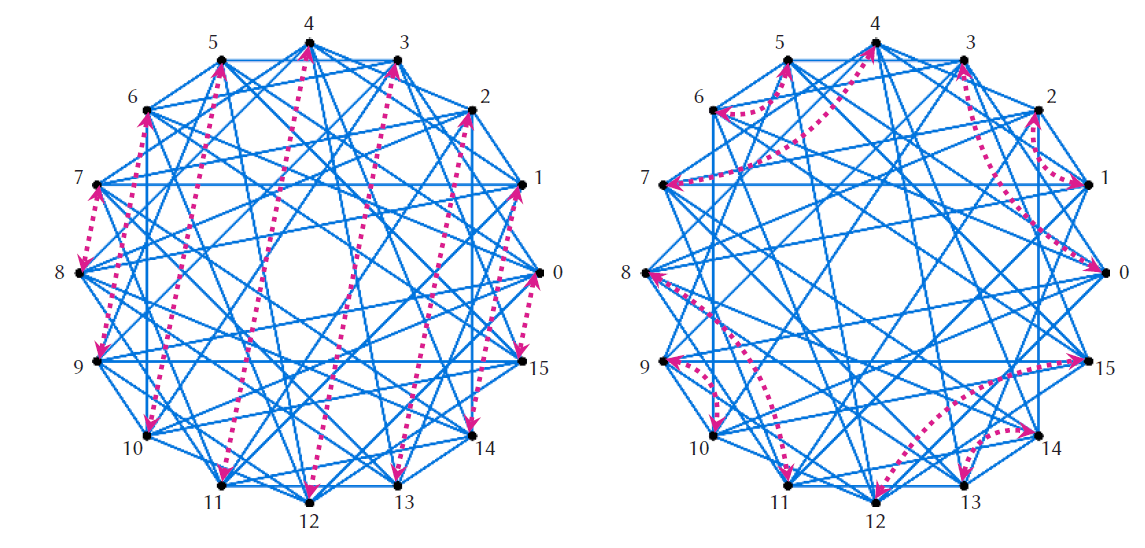Symmetry with respect to swapping $x$ with $x\oplus (2^n-1)=15-x$ (left), and $x$ with $x\oplus 3$ (right).

## Where can we go from here?We have unlocked some, but by no means all of the mysteries presented by images like the Hamming fractal, and circle visualisations. There are many symmetries left to uncover, and fascinating questions to ask about these images. Why is there a white circle at the centre? How is the size of this circle related to $n$ and the chosen Hamming distance? Why does there seem to be more white space toward the centre of the circle pictures? What can be said about the structure of the white space around the centre circle, for example the eight ovals surrounding it?While it is not at all obvious from the circle picture for $n=4$, $k=2$, this graph actually has two connected components shown on the right. Is it always the case for these graphs? If not, under what conditions is it the case? Can the number of connected components be described in terms of the numerical properties of the vertex numbers they contain? When do these graphs have other interesting properties; for example, when are they bipartite? Just two examples to illustrate the variety of circle virtualisations are below.

The circle visualisations for $B_7$ if $H(i,j)=5$ (left), and $B_8$ if $H(i,j)=2$ (right).

The Wolfram demonstration projects by Enrique Zeleny (2014) and Chris Boucher (2020) are freely available and offer a platform for quickly generating some of these images. I hope you share my enthusiasm for these pictures and will feel inspired to explore them yourself.# Slaying the dragon

Against all the odds, your plan has worked. Your thief snuck in ahead of the team and got a surprise backstab, the paladin’s shield deflected the would-be deadly dragon’s fire breath and a well-aimed wizard’s frostbolt took out the columns of the building. You move your dwarven barbarian in for the killing blow. The trapped red dragon looks up with anger in its eyes. You know, all that stands between this creature and death is a single good hit of your axe. Even with all the work from the other players, the difficulty class of landing a hit on this creature is 16, meaning you will only hit it with a roll of 16 or higher on a d20 (a 20-sided die for those not in the know). That’s a $1/4$ chance of hitting.

Luckily, there is one last hope. The druid spent their turn giving you the help action. This means your next attack has advantage. Advantage allows you to now roll two d20s and choose the highest of those two values. This is obviously helpful, but what are the new odds that you will be able to give this dragon a one-way ticket to the afterlife?# My secret

Mathematics is my secret—
my secret weakness.
I feel like a stubborn, helpless fool in the middle of a problem.
Trapped and crazed.
Also, thrilled.
I’m gnawing on a bone and I fear
for anyone who comes near and tries
to make me stop.
I growl. I refuse to drop that bone.
I am dogged—
did I mention that math is funny too?
It’s hilarious how much time I secretly waste on it,
when there are more productive things to do,
like walk my dog,
fold my laundry,
or finish my actual homework.

No one in my family thinks all this math is healthy.
Get some sleep.
Here, eat something.
Go outside for some fresh air!
And they’re probably right.
But here’s another secret:
I don’t care
About rest or nutrition or any of it.
blah
blah
blah
I’m not even really listening.
Too busy gnawing away.

On a different day
Back out in the blaring, glaring, glittering world
I feel unfurled and chatty
Eager for random conversations and observations
Nosy for other people’s secrets, which surely must be
as weird and wild as the ones that define the inside of me.
And I notice
something fascinating
exasperating
in all the talking
talking
talking.

Here’s what a lot of people say:

I’m just not a math person.
Insert shrug and sheepish smile.
I can barely figure out the tip.
Wait, you take extra math classes? What for?
What do you mean, just for fun?
Wow, you must be a genius or something.

Here’s what no one says:

I’m just not a words person.
Insert shrug and sheepish smile.
I can barely read a sentence.
Wait, you read an entire book this weekend? What for?
What do you mean, just for fun?
Wow, you must be a genius or something.

I feel silly being mistaken for a genius,
when I’m really just a nerd.
Struggling,
stumbling,
pushing,
reaching
for more
of something
that makes
me feel
mesmerised and uncomfortable,
lost,
hopeless,
hopeful,
real.# Covering infinity

I’m sure some of you reading this may know about topology vaguely as “that one where the doughnut is the same as the mug”. And you would be right. In essence, topology is maths after you have forgotten what distance is, meaning that space is squishier than we’re used to. Then, the only defining feature of a mug is the hole between its body and its handle and the only defining feature of a doughnut is… well, its hole. A lot of topology is about saying whether one shape is the same as another (such as doughnuts and mugs).

Today we’re going to be going a step further; to ask when two shapes look the same ‘up close’, despite not necessarily being the same overall. For example, if we zoom in closely on any part of a circle, it will look like a line. However these shapes are not the same, since a circle is not a line. We’ll discuss this in more detail soon.

The question I want to answer is whether there is a way to intuitively create a shape that ‘covers’ another. I put it to you that there is. If shape $A$ covers a base shape $B$, there must be a way to map $A$ to $B$ such that

• the base $B$ is entirely covered by $A$ (ie this is a surjective map); and
• by zooming on any small patch of $B$, the map looks like the identity map.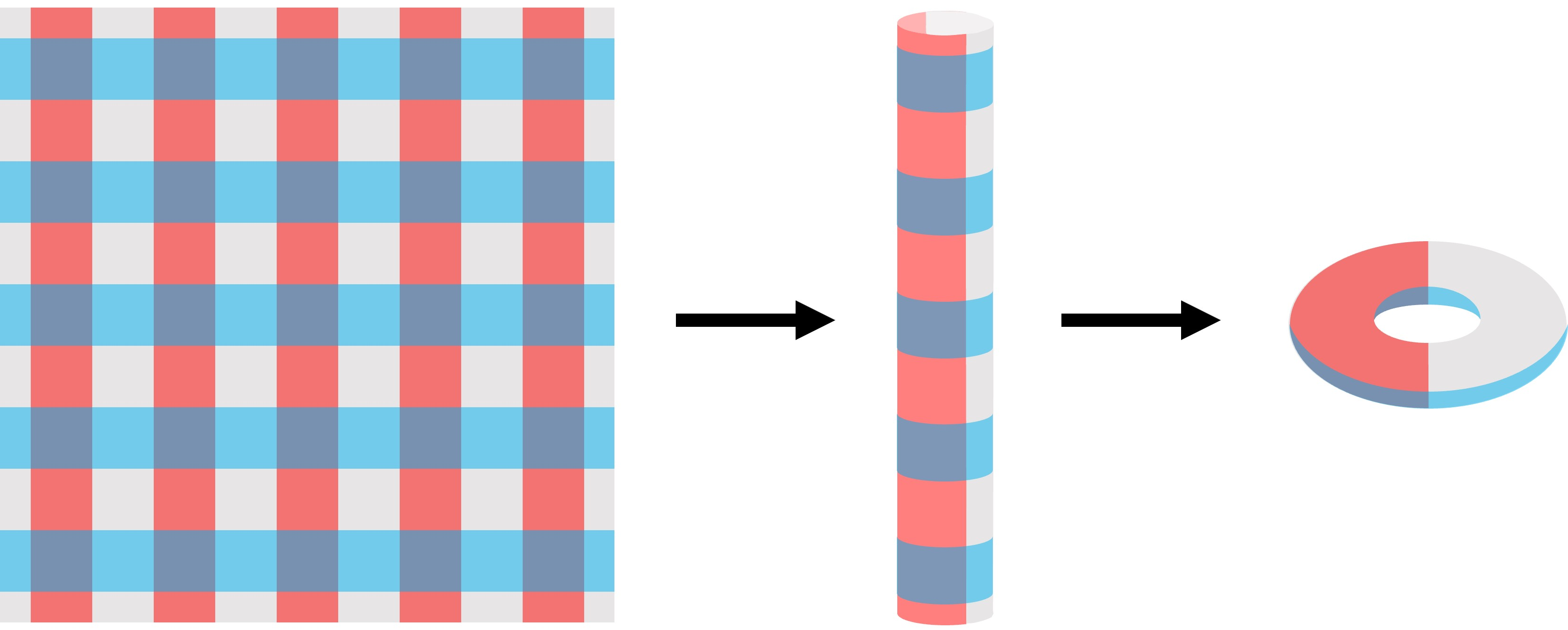The infinite plane covers the torus: the colouring has been added to illustrate how the covering map works.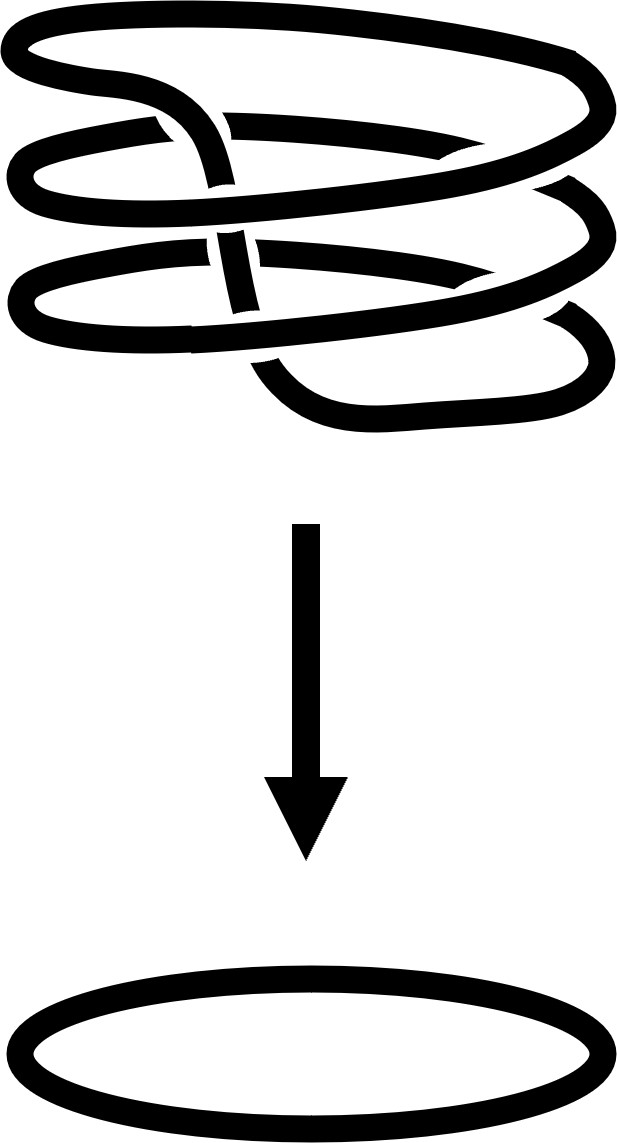For instance, we can say that a plane covers a torus. To visualise this, imagine taking the plane, rolling it up into a cylinder, then rolling down the edges of the cylinder, sort of like rolling down a sock. As the edges loop round, the resulting surface is—lo and behold—a torus! This way, every part of the torus is covered (satisfying surjectivity), and every small part of the plane looks like the surface of a torus if you zoom in close enough.

Another example of this is the circle. Any circle can cover another circle by winding round it some number of times. You can imagine that this is like wrapping an elastic band around your finger. Above on the right we have a circle mapping continuously onto a circle a third of its length.

But we can think bigger; the real number line can cover a circle by twisting it round into a helix.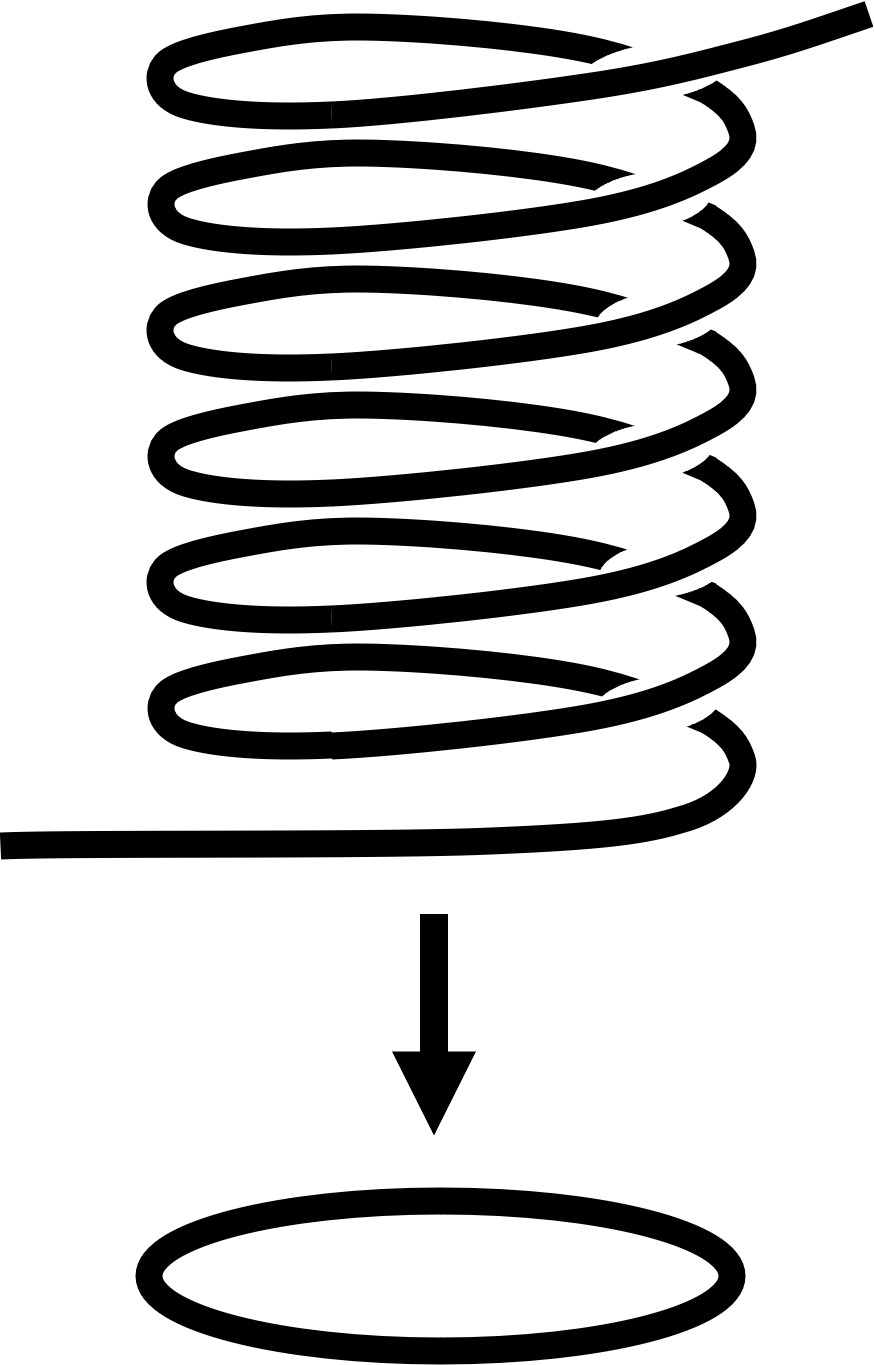This satisfies surjectivity since every part of the circle is covered, and, as mentioned above, every part of the helix looks like a circle if you zoom in close enough. However, a finite interval cannot cover a circle since if we zoom in on the interval’s endpoints, we can’t find any point on the circle that looks like this.

Don’t worry though, our bounded interval can still play with other bounded intervals.

## Infinity undercoverSo, we’ve seen how to cover lines and circles, but how does this covering business work when lines intersect? The infinity symbol, $\infty$—since I always like to be able to call a symbol something in my head, we’ll pronounce this ‘infty’—has two edges intersecting at one vertex.

This is an example of a graph: a shape consisting of vertices and edges. Each vertex in a graph has a valency, ie the number of ‘arms’ it has. So if two edges meet at a vertex, they become four ‘arms’, and the intersection is a point with valency 4.

Now we can reinterpret the way we think about covers; a circle cannot cover infty since zooming in on the infty at the intersection we have a point where four lines join together, whereas no point on the circle has this property. One idea is to cover this with another infty, using the identity map. But, to make other covers, let’s look at more graphs that are 4-valent at each vertex.

To make sure that we are not mixing up the two arms of each edge, let’s give each edge a direction so we can easily differentiate the ‘source’ end of the edge and the ‘target’… end. Also colouring the edges is a simple way of making sure that we distinguish the two different edges.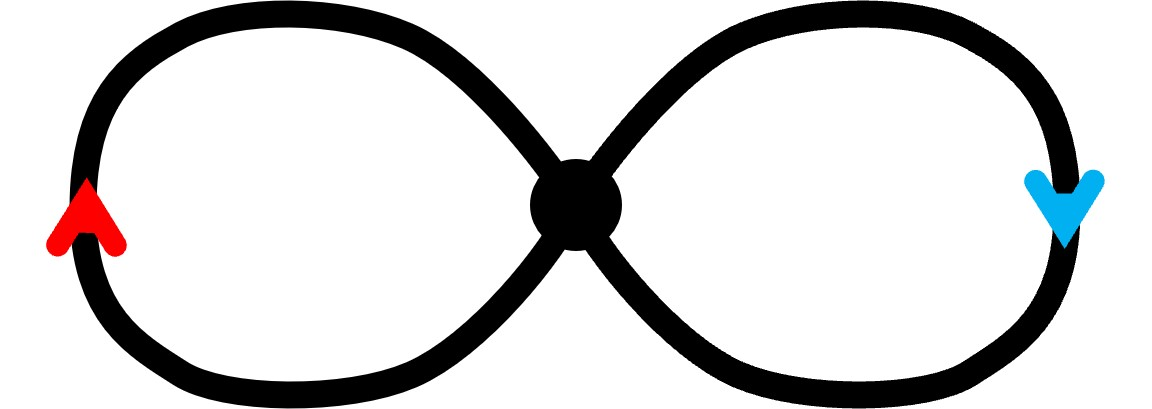A constraint for a graph to cover infty is that every vertex in the cover must have two red arrows (one source, one target) and two blue arrows (likewise). Let’s call the graph with arrows a directed graph, or a digraph for short. An example of such a cover is this: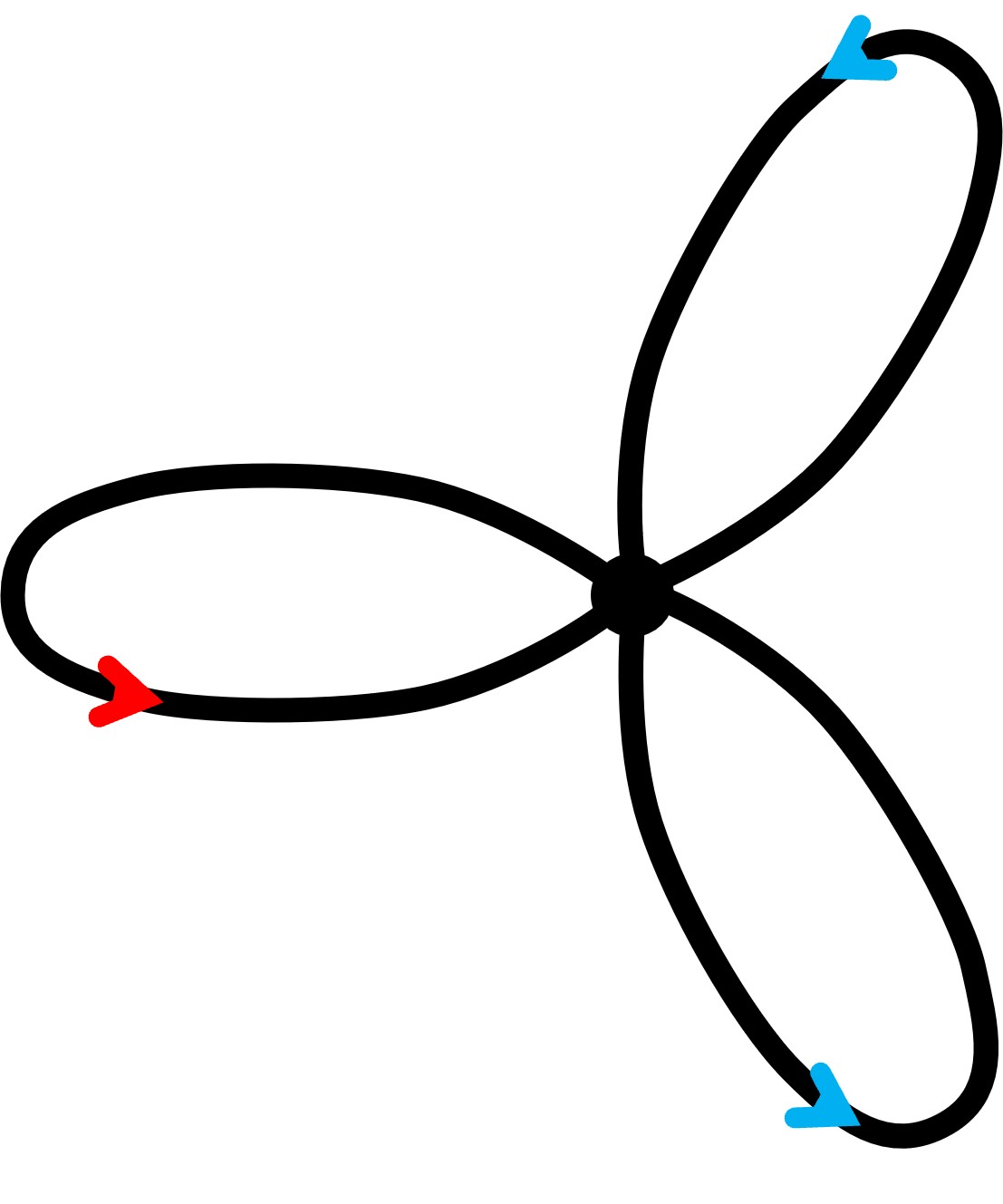From this we get infty by double-looping the blue-edged loop so that the two vertices end up on top of each other, with red edge on red edge and blue edge on blue.

An example of a case where this does not hold is on the right. This does not work for a few reasons. You might have noticed that its vertex has too many arms, or that there is more than one blue arrow pointing towards/away from the vertex. Is there some other way to colour and direct the graph to make it a cover of infty? Well, whichever colouring we choose, we will always have more than two of one colour. So sadly, I topologise: this graph is not destined to cover infinity.

## Inftys all the way down

Another cover is the infinite grid. A way to conceptualise this map is thinking similarly to the example of the plane rolling up to make the torus. First we roll the graph into a vertical cylinder, then we roll the edges of this cylinder down so that each rung is mapped onto the same one.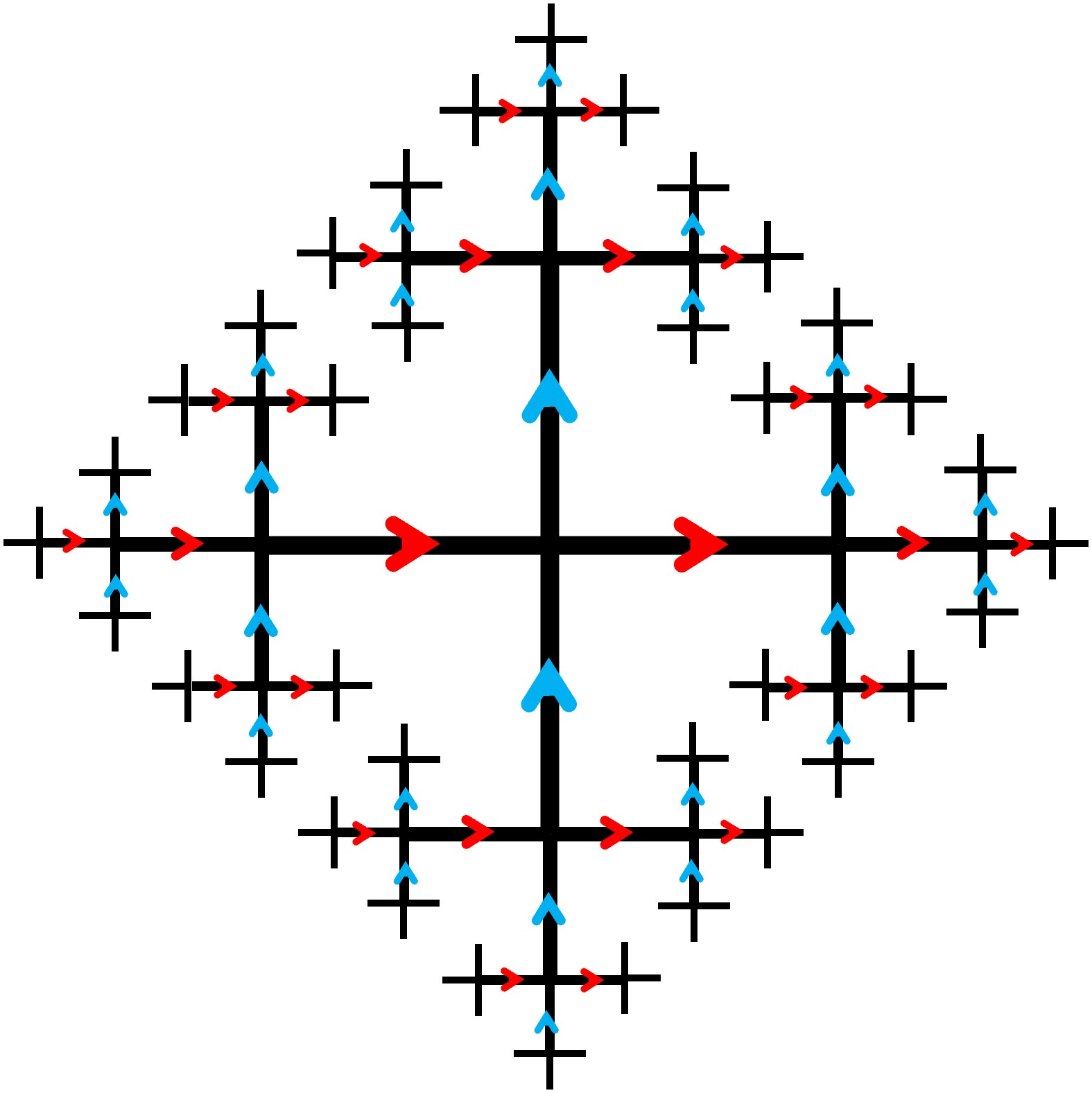Up until this point, taking a red path then a blue path in each cover will end up at the same point as taking a blue path and then red (try this out on the infinite grid). But can we break this pattern?

Here we find a special case I call the fractal cross, where the cover is so intricate that the path between any two vertices is unique. Try starting at any vertex on the graph, and mark out the path travelling red then blue. Now go back and do the same with blue then red. Your destination depends on the order of your steps! I call this the fractal cross because it is infinitely detailed—like a fractal. The fractal cross covers infty in a similar way to the infinite grid. This, *drum roll please*, is the largest cover of infty. More precisely, it covers every other cover of infty and nothing else covers it.

## From cross to cover

All covers of infty can actually be derived from the fractal cross. As an example, if we take the central blue line of the graph, we can first wrap it around itself in the way that a line covers a circle; and then glue together all the branching red arms, and we will get this cover: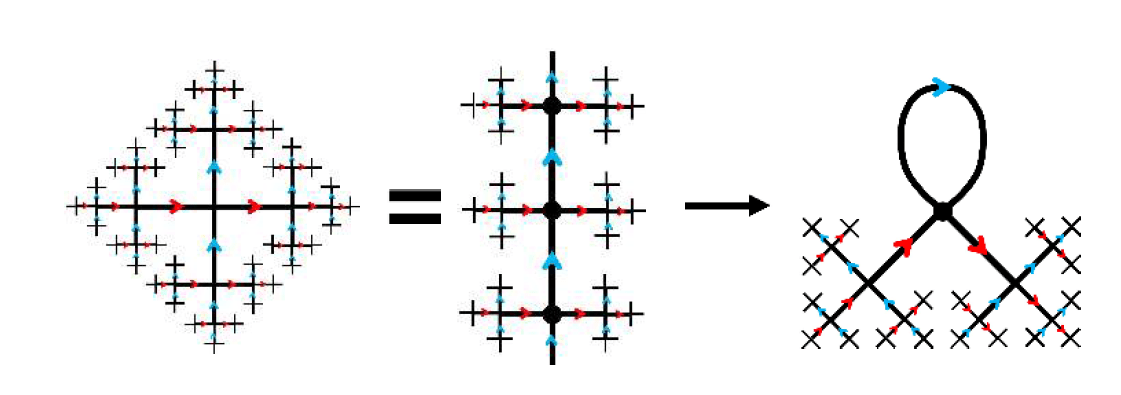By repeatedly gluing and warping the fractal cross we can find more covers, and eventually get down to infty.

For instance, let’s again take a blue-edged line on the fractal cross, but this time wind it around until every third blue edge in the line is glued together creating a loop. Now do the same with a red-edged line intersecting this loop. Next glue the vertices of the loops together one by one. As you can see, this forms a triangle with vertices intersecting a circle. Finally this graph with six edges collapses down onto infty.## Illuminati confirmed?

Every cover we’ve looked at so far has been 4-valent; in fact we have now recognised the 4-valency of every vertex in the cover as a necessary condition to be a cover. But is it a sufficient condition? If we have a 4-valent graph, will it necessarily cover infinity?

We can generate complex, and often infinite covers of infty, all just by checking all our rules are satisfied. In this way, we have a surprising amount of flexibility to build up such a cover. You might enquire further; how much flexibility? Can we explore some artistic licence?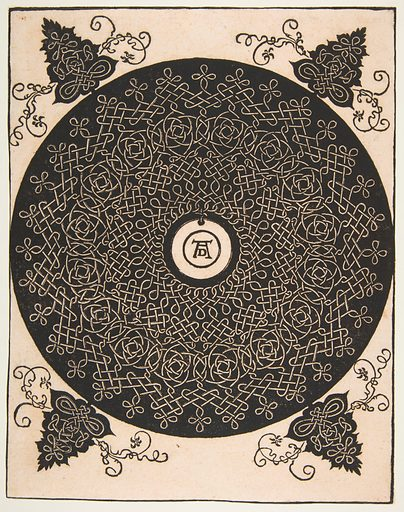For example, would a Celtic knot cover infty? A Celtic knot is a traditional weaving pattern, which is drawn like a rope tied in a knot, with the notable property that the two rope ends are stuck together. If we shine a torch on such a knot and take a picture of its shadow, we can imagine that the resulting silhouette is a graph. All vertices of this shadow graph will be 4-valent since it is the junction of two lines crossing. So… does this cover infty?

A beautiful Celtic knot designed by Leonardo da Vinci is shown on the right. Below, I have drawn the digraph associated with the centre piece, verifying both that it indeed covers infty, and that I have far too much time on my hands. In fact, we find that a Celtic knot’s shadow will always qualify as a cover of infty. This is to say that there will always be a digraph of the knot satisfying the requirements—each vertex having a blue source, blue target, red source, and red target.

For the interested reader, the fact that the shadow of any knot diagram can be made to cover infty follows from a few neat ideas in knot theory. First, the shadow of a knot diagram is, by definition a planar graph, and it is possible to ‘chessboard colour’ the complementary regions of this graph, ie colour those regions black or white so that no adjacent regions have the same colour. (There are several ways to prove this, one way being to prove that the graph ‘dual’ to the complementary regions contains no odd-length cycles by the definition of a knot.) Now choose an orientation of the knot, which gives an orientation of each edge in the shadow graph. Assign an edge the colour red if the region on its right is black, and blue otherwise. In this way the graph can be directed and coloured, and it is straightforward to check that every vertex has four arms, two red and two blue, and one incoming and one outgoing of each colour. This means the graph covers infty. In fact one can even prove that every regular 4-valent graph covers infty, but this is trickier, using the so-called 2-factor theorem.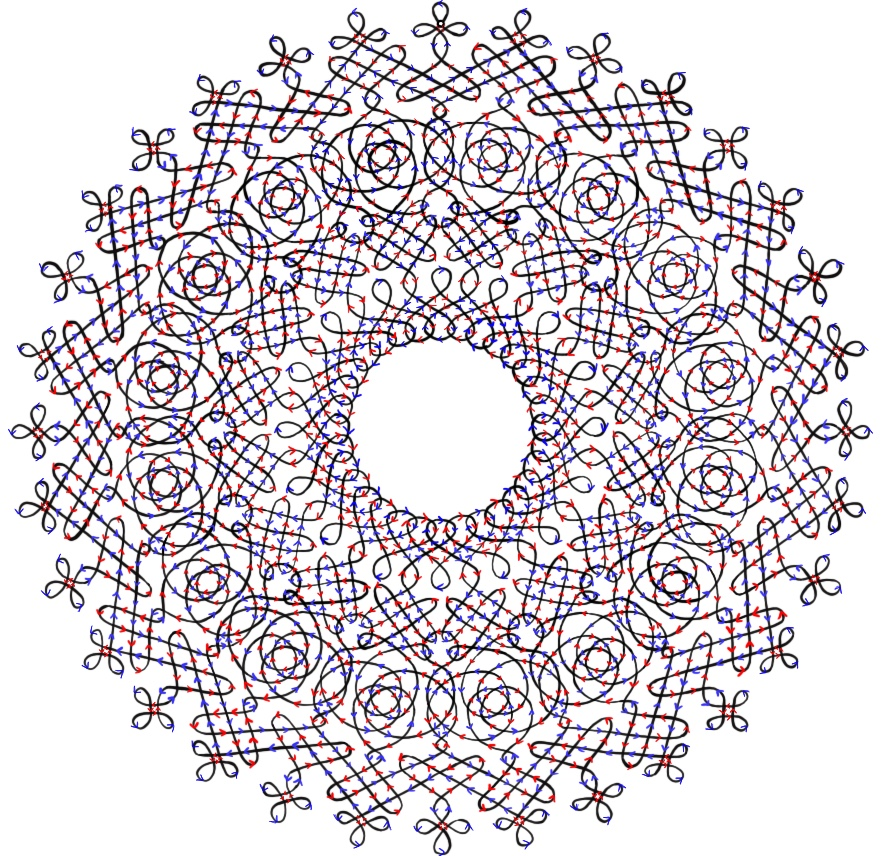It is fascinating that an artistic piece could relate to such a mathematical idea as a topological cover, and all of this stemming from the theory of a torus being considered topologically identical to a mug—who would have thought? The realisation that all knots (apart from the circle) cover infty is non-trivial and—in my opinion—really cool. However, as cool as topological space is, just make sure you don’t try pouring any tea in your doughnut!